# R S Aggarwal Solutions for Class 10 Maths Chapter 15 Perimeter and Area of Plane Figures

R S Aggarwal solutions for class 10 Chapter 15 helps students to solve all the questions on the topic Perimeter and Area of Plane Figures such as circle, triangle, semicircle and rectangle. Detailed solution of each question is provided here so that students can compare their answers to the sample response. Some tips and tricks are also suggested by subject experts here. Download and practice Chapter 15 of R S Aggarwal Class 10 textbook to know more about plane figures.

## Download PDF of R S Aggarwal Solutions for Class 10 Chapter 15 Perimeter and Area of Plane Figures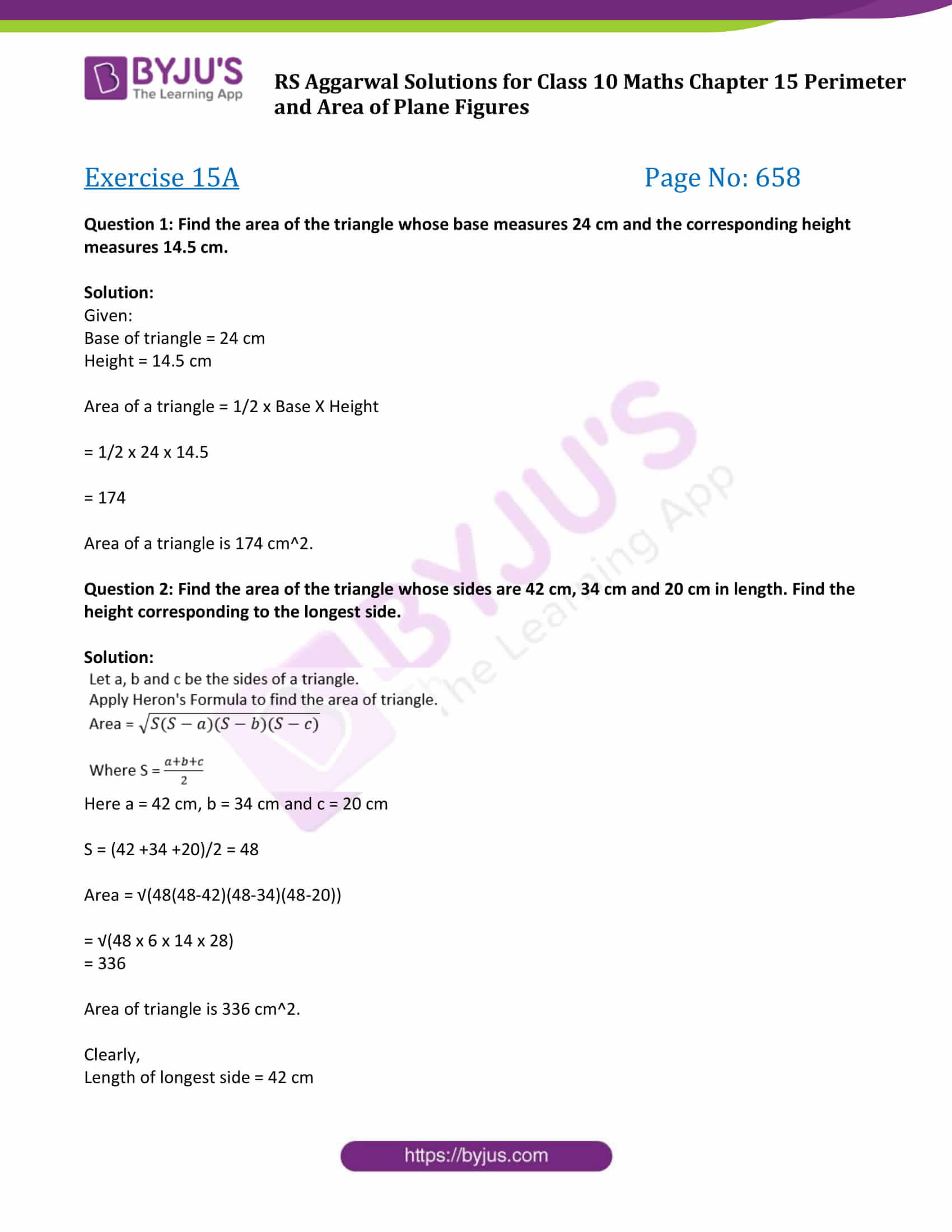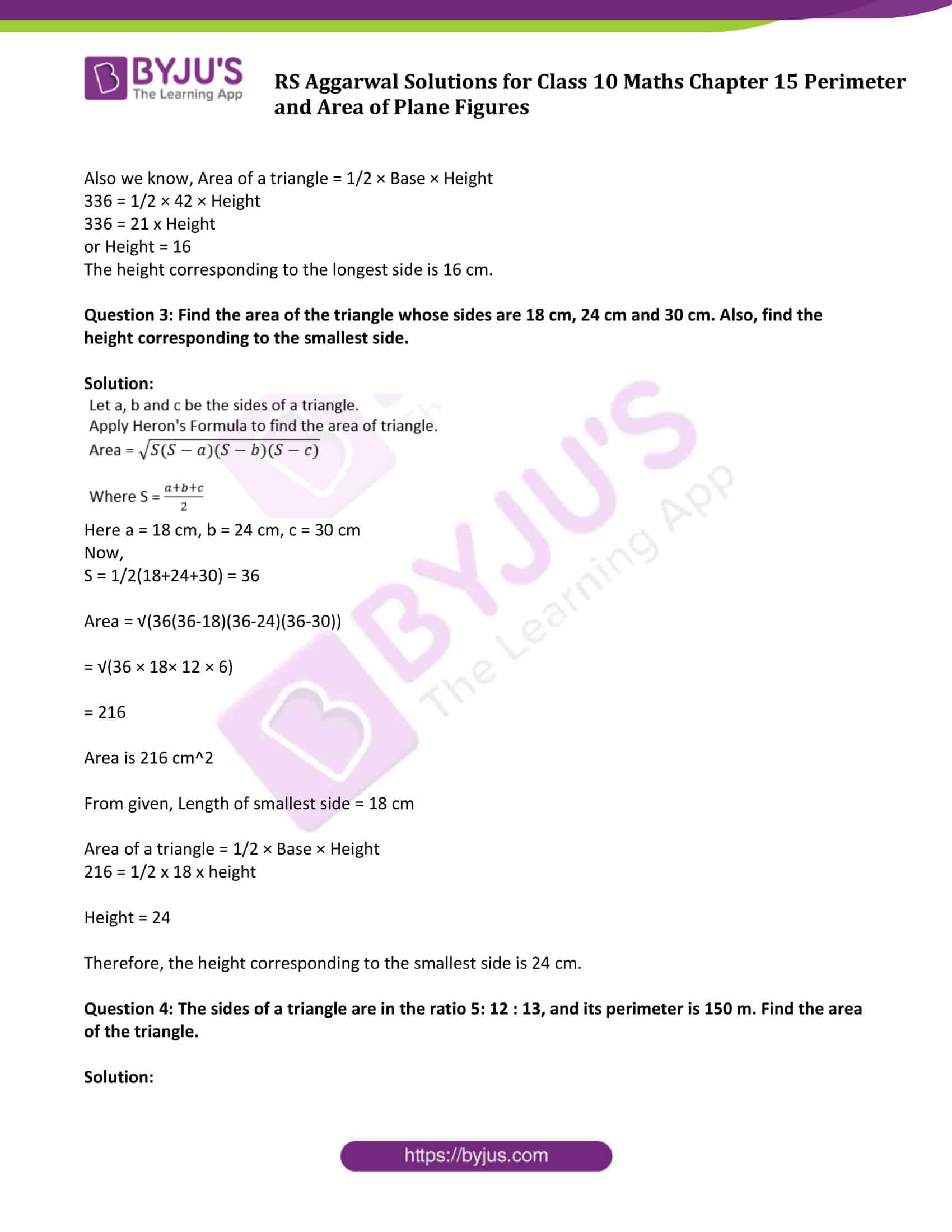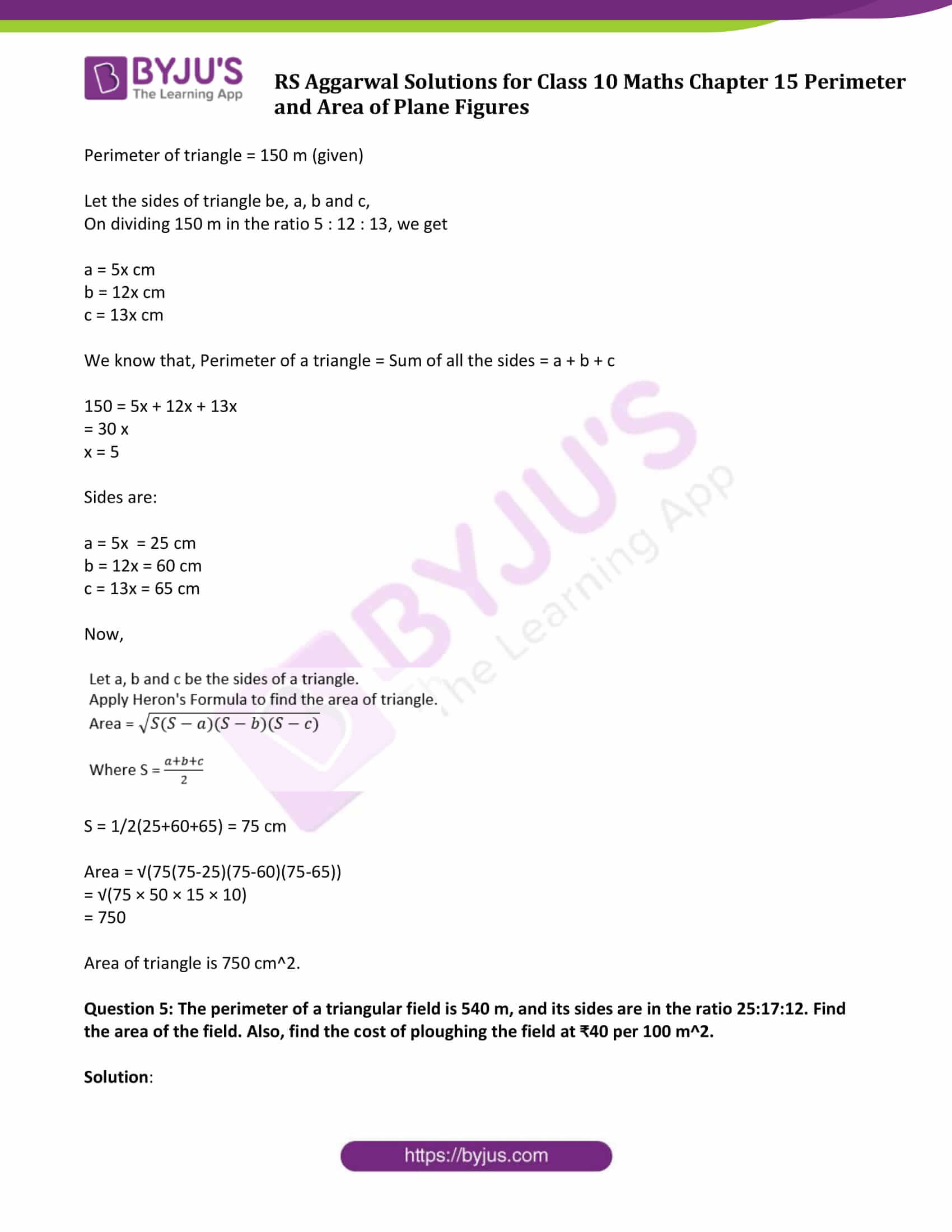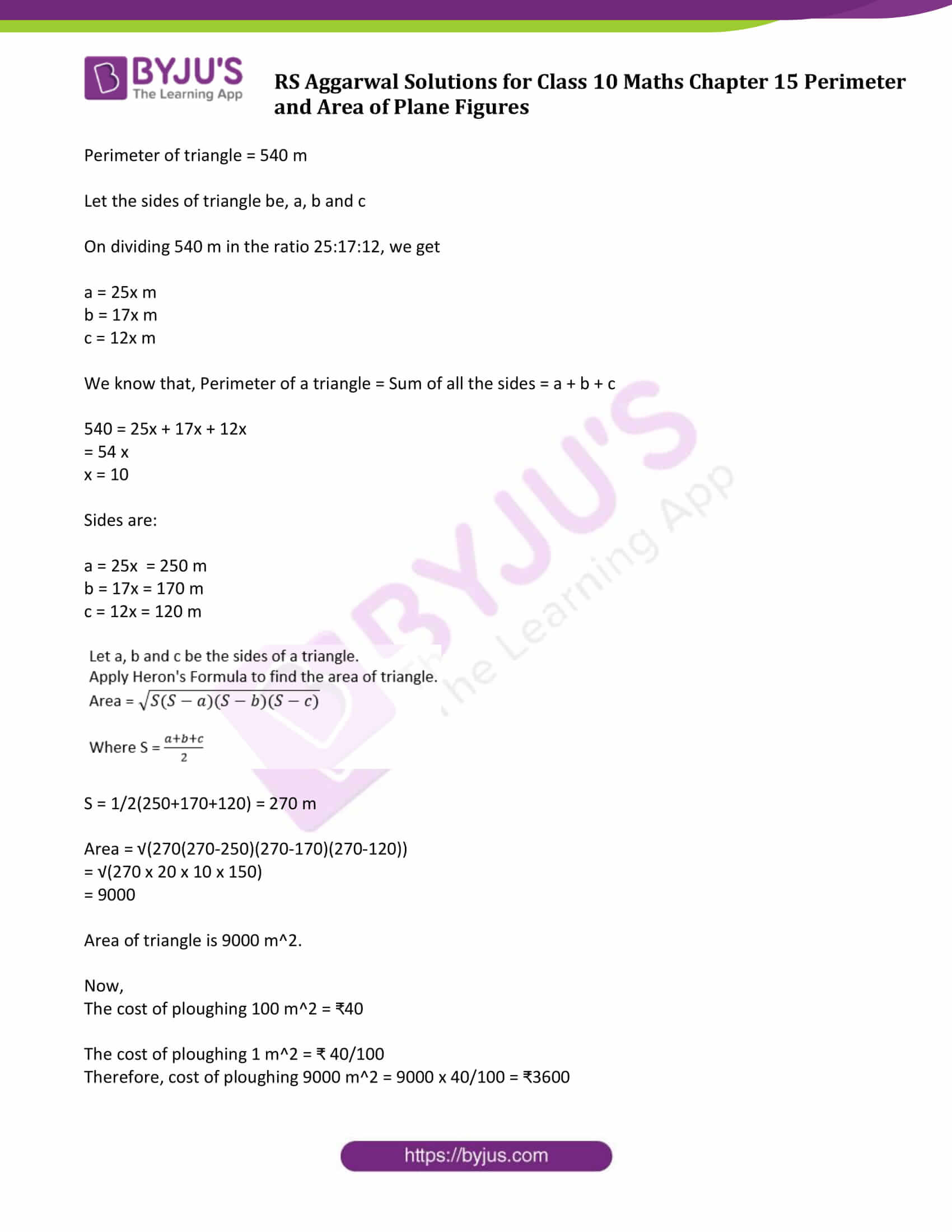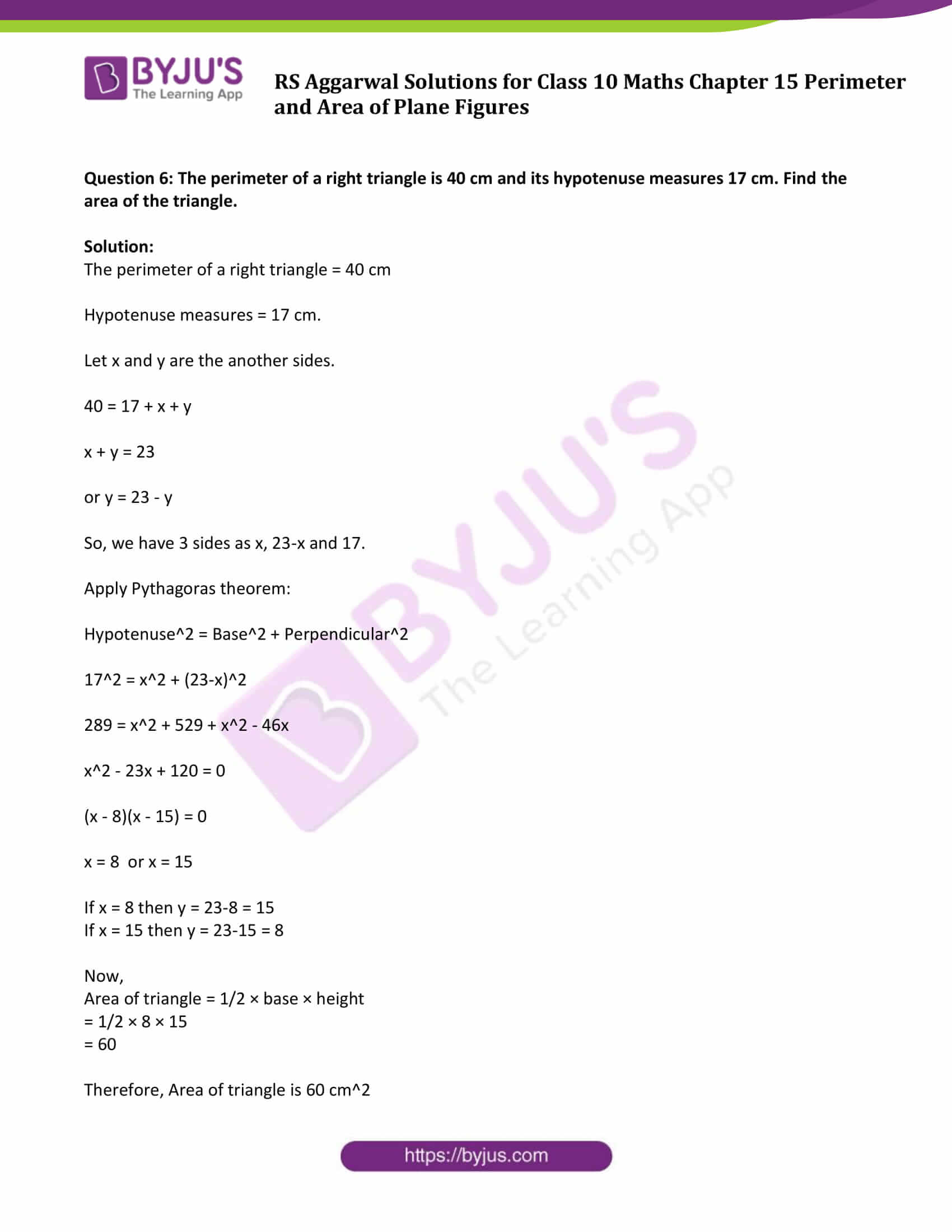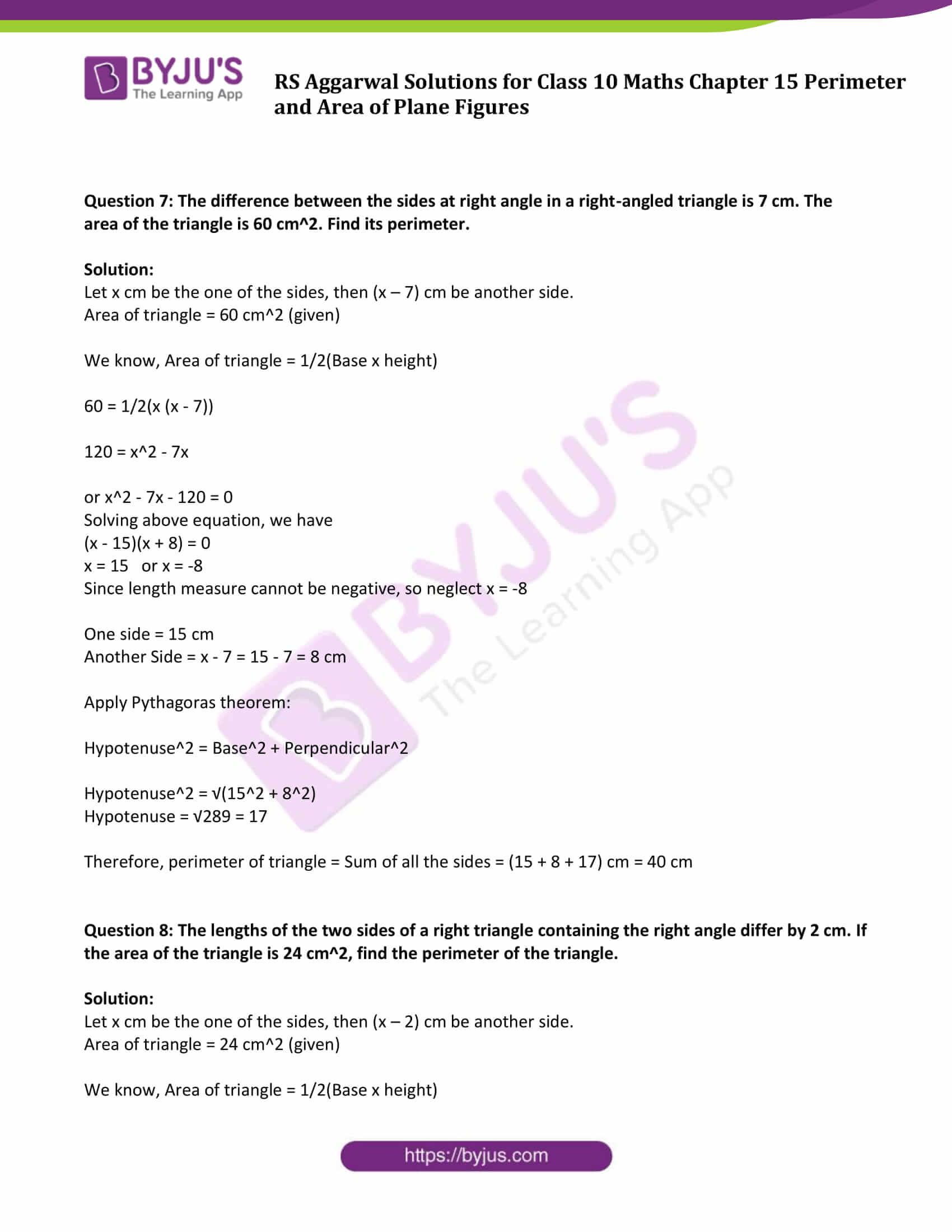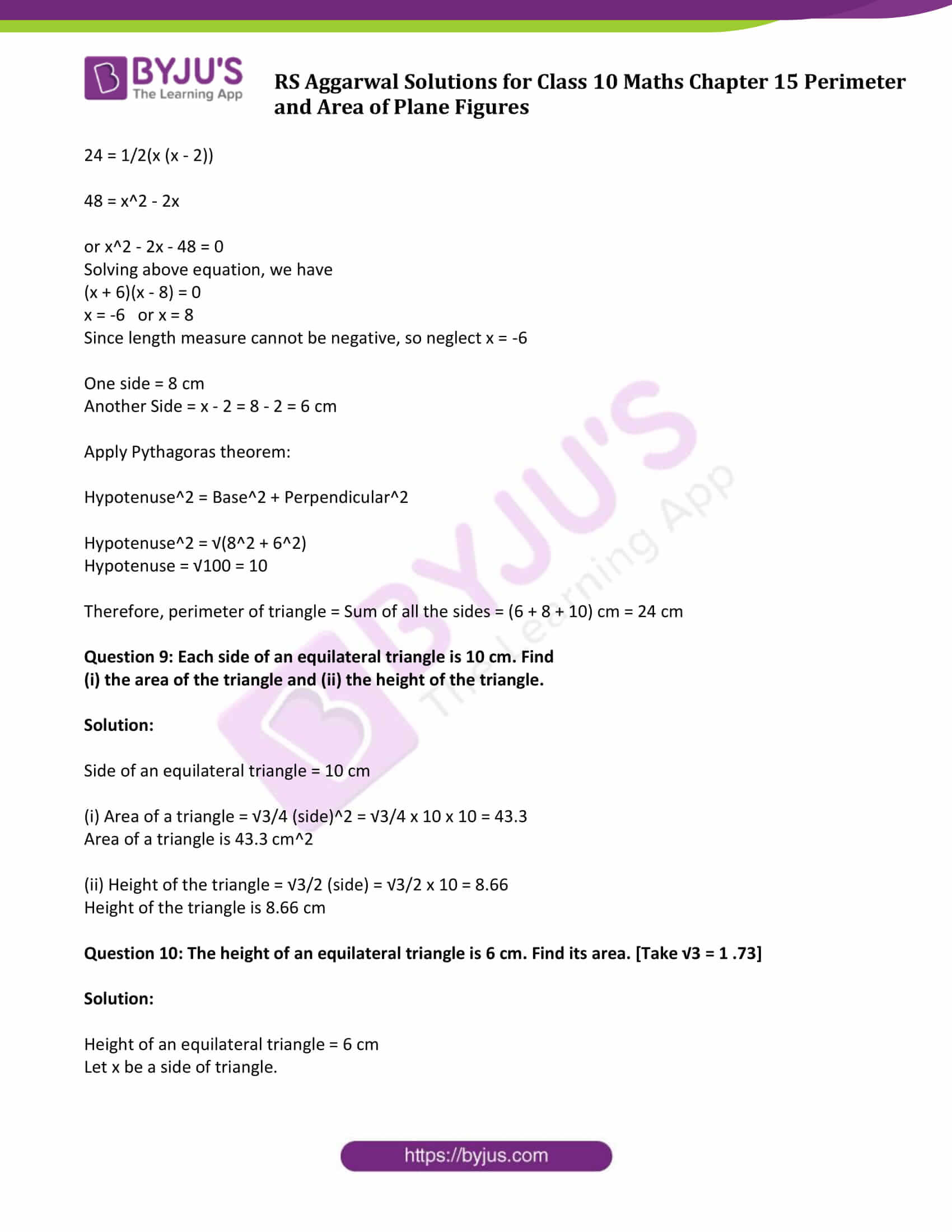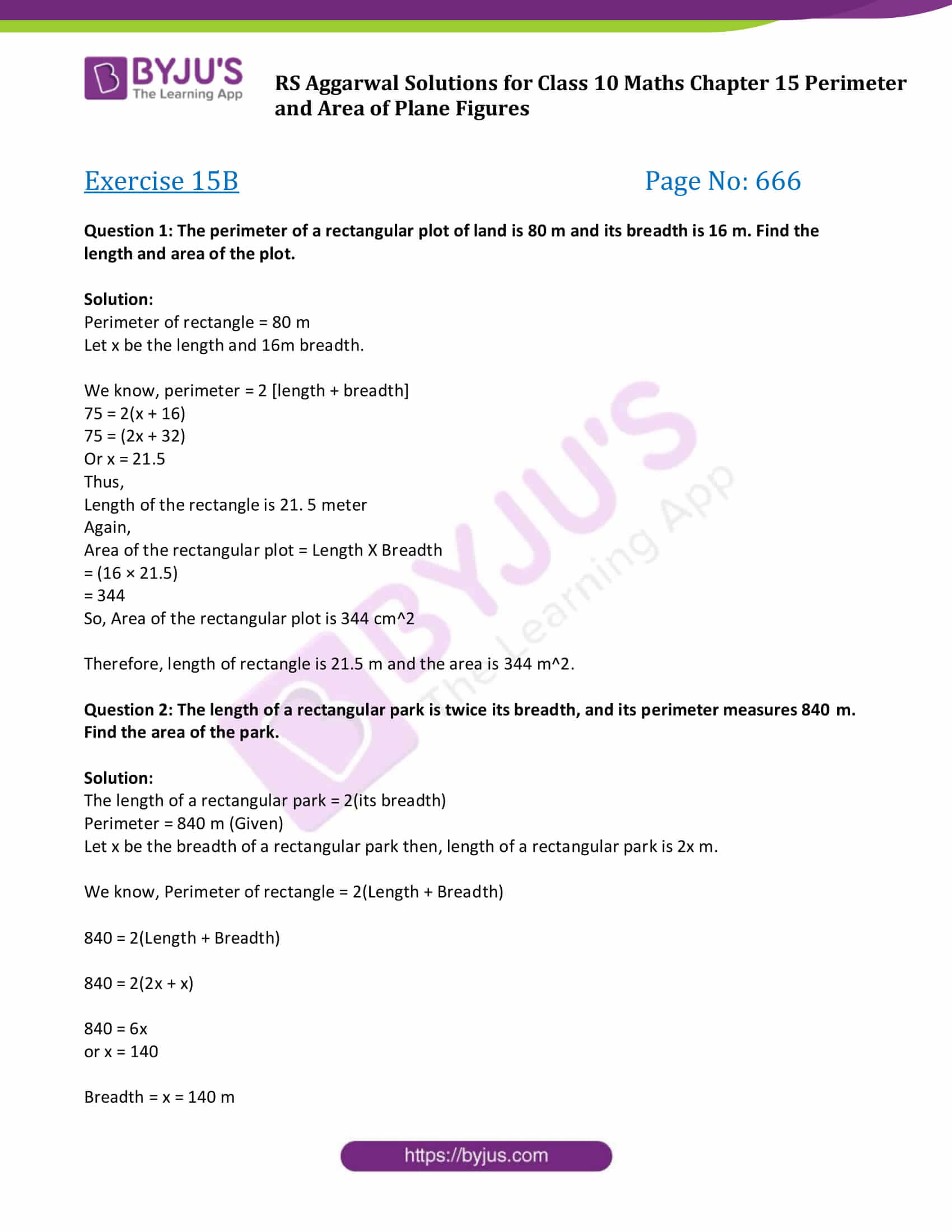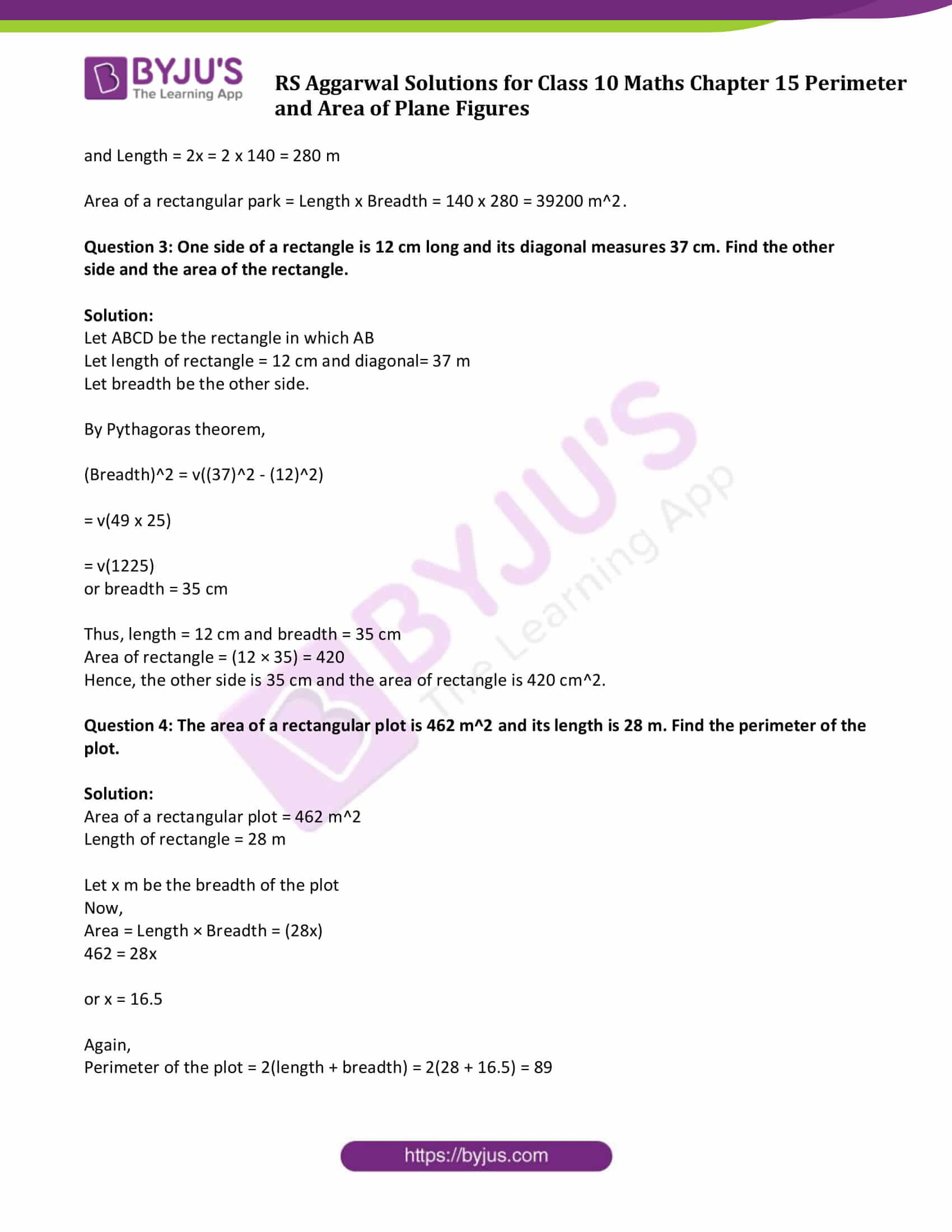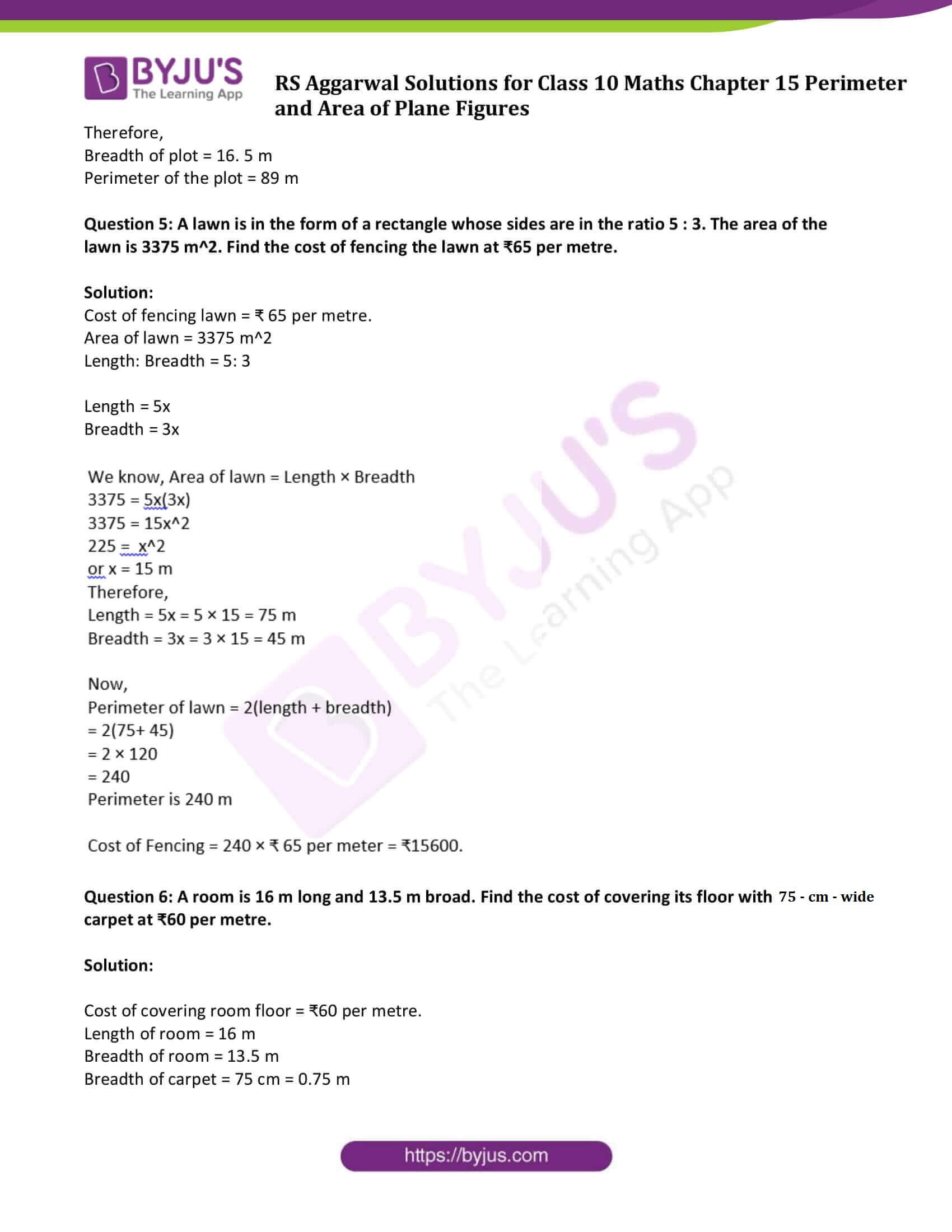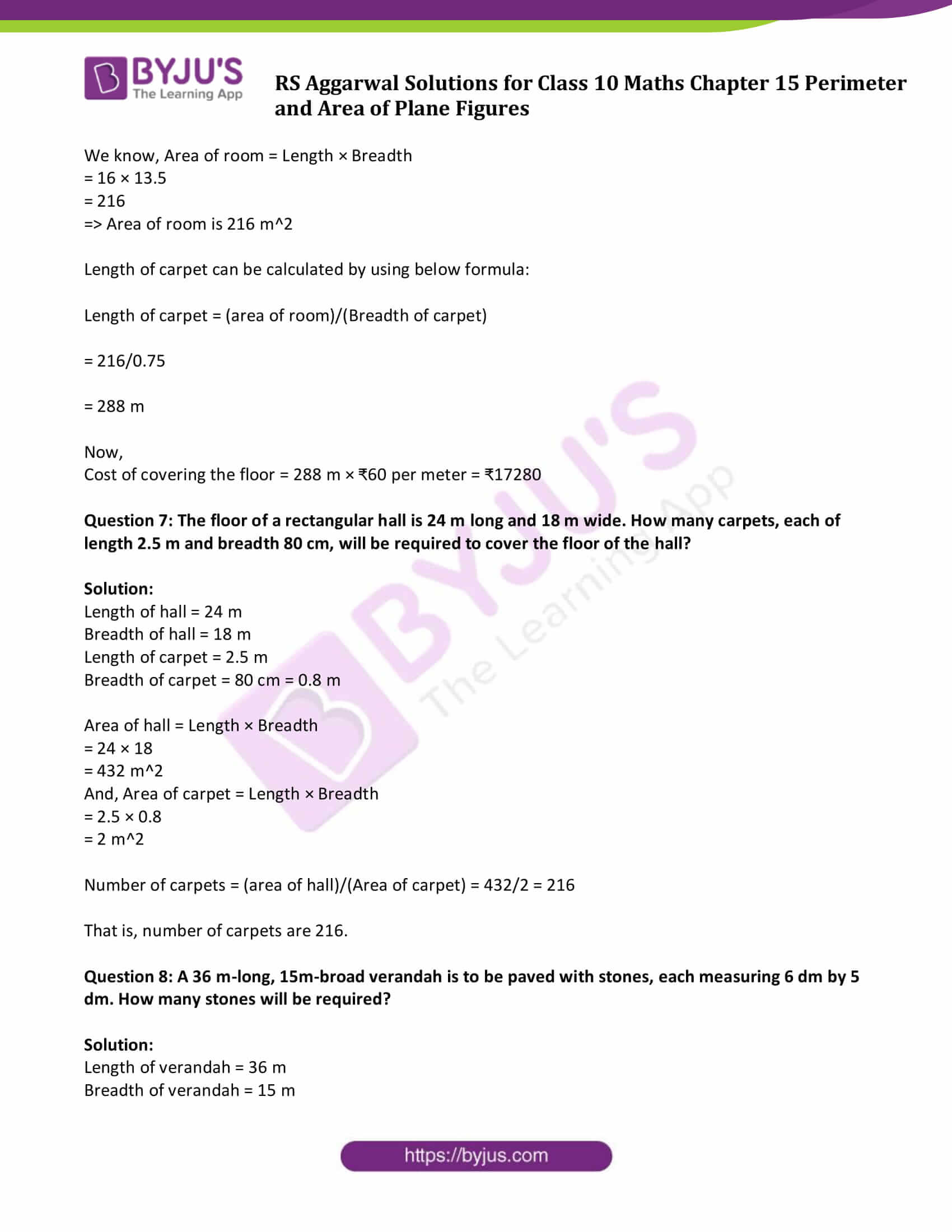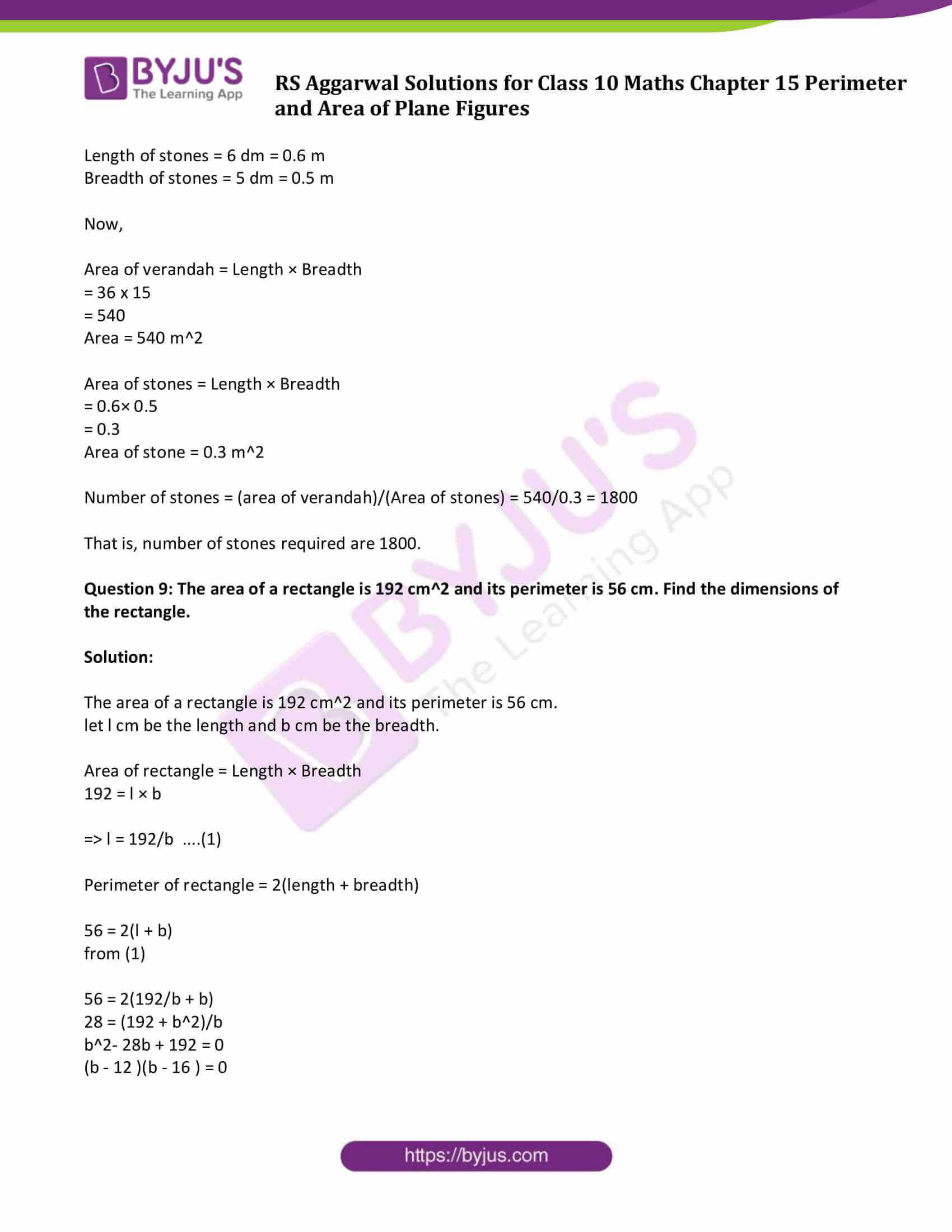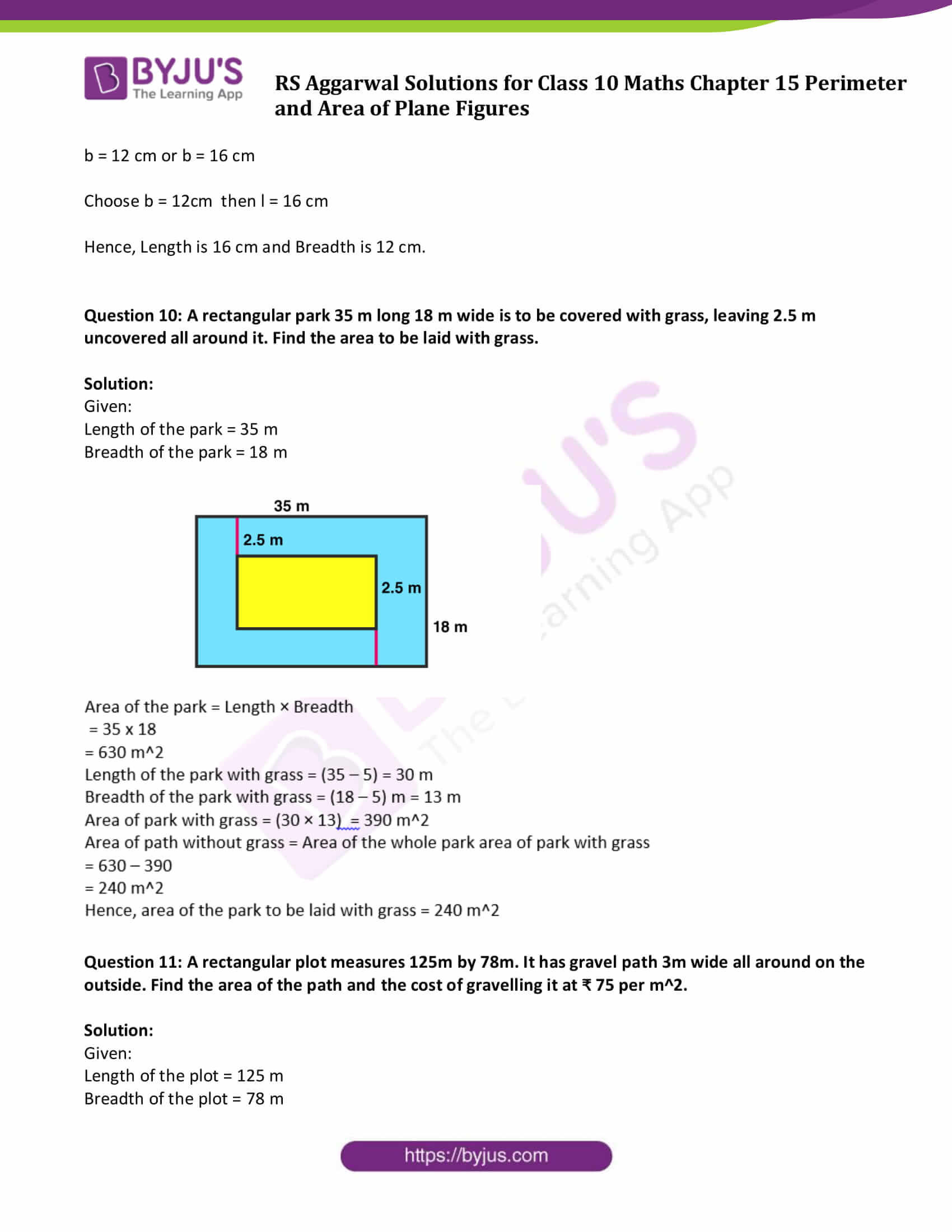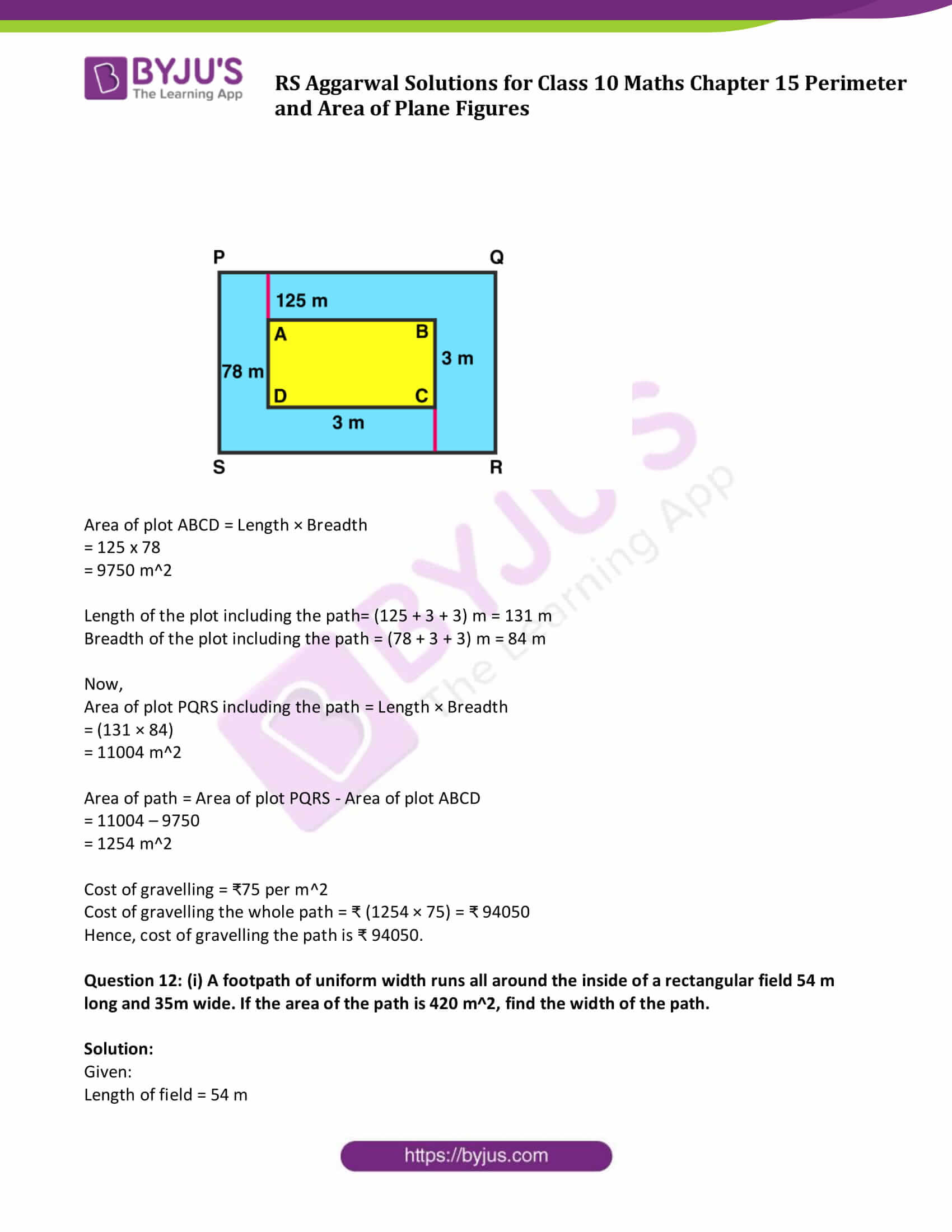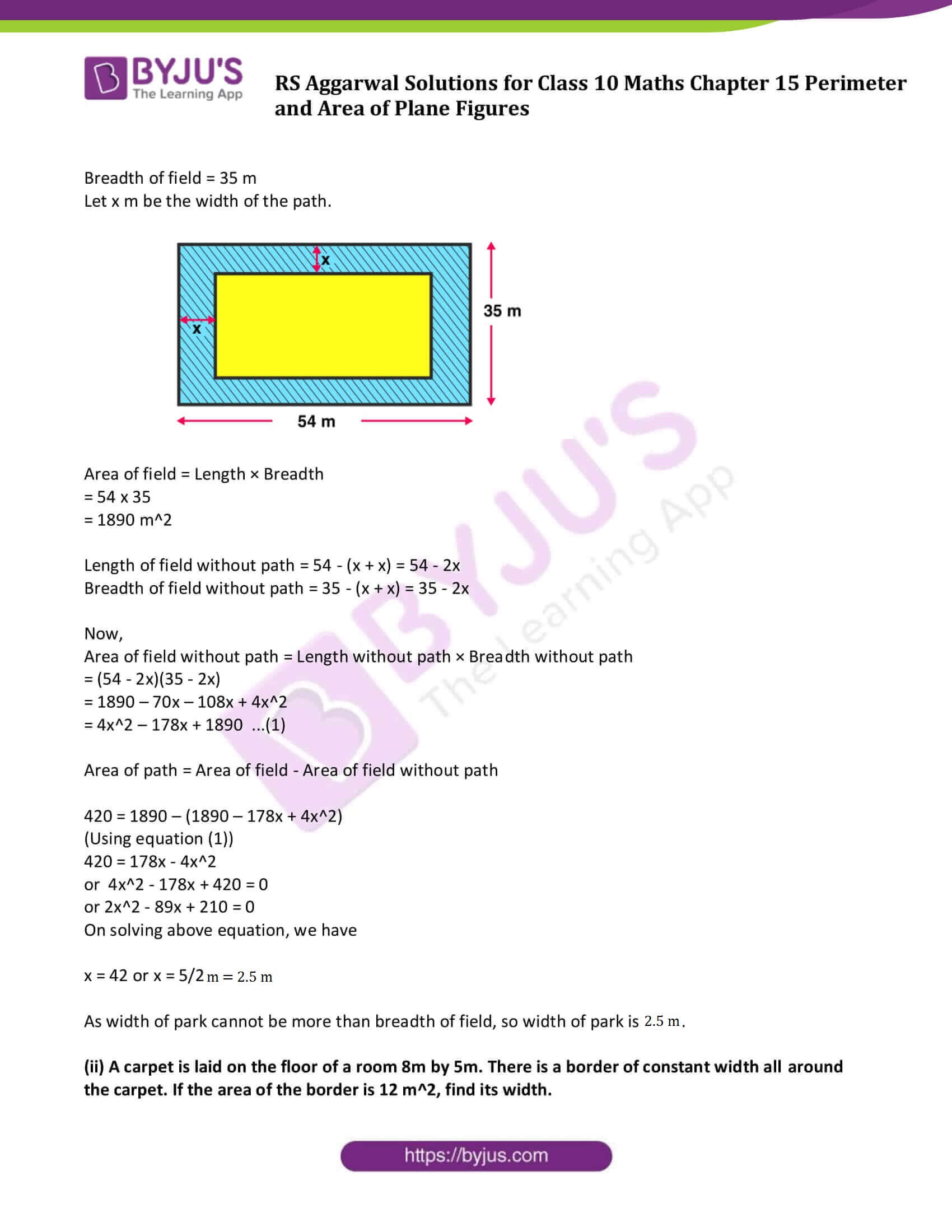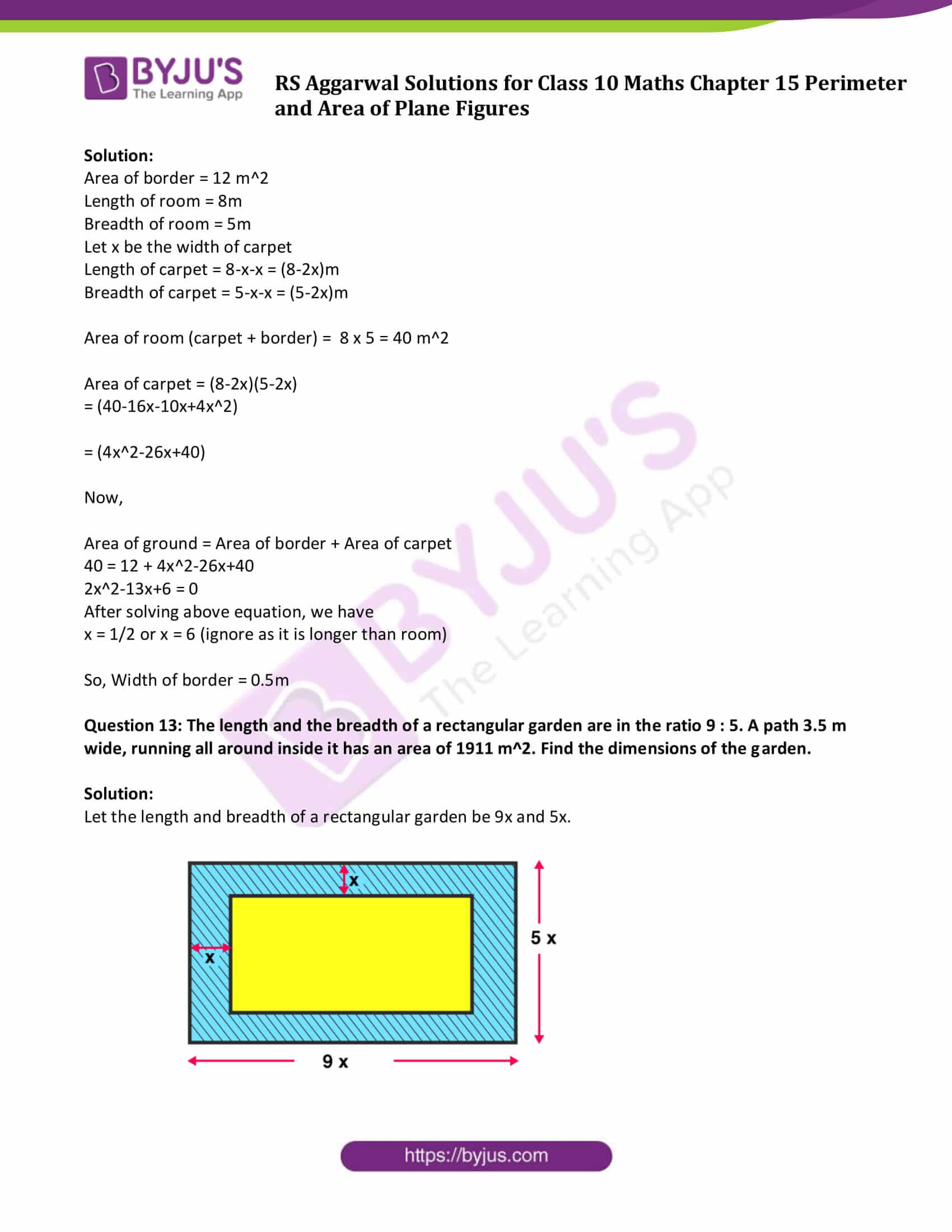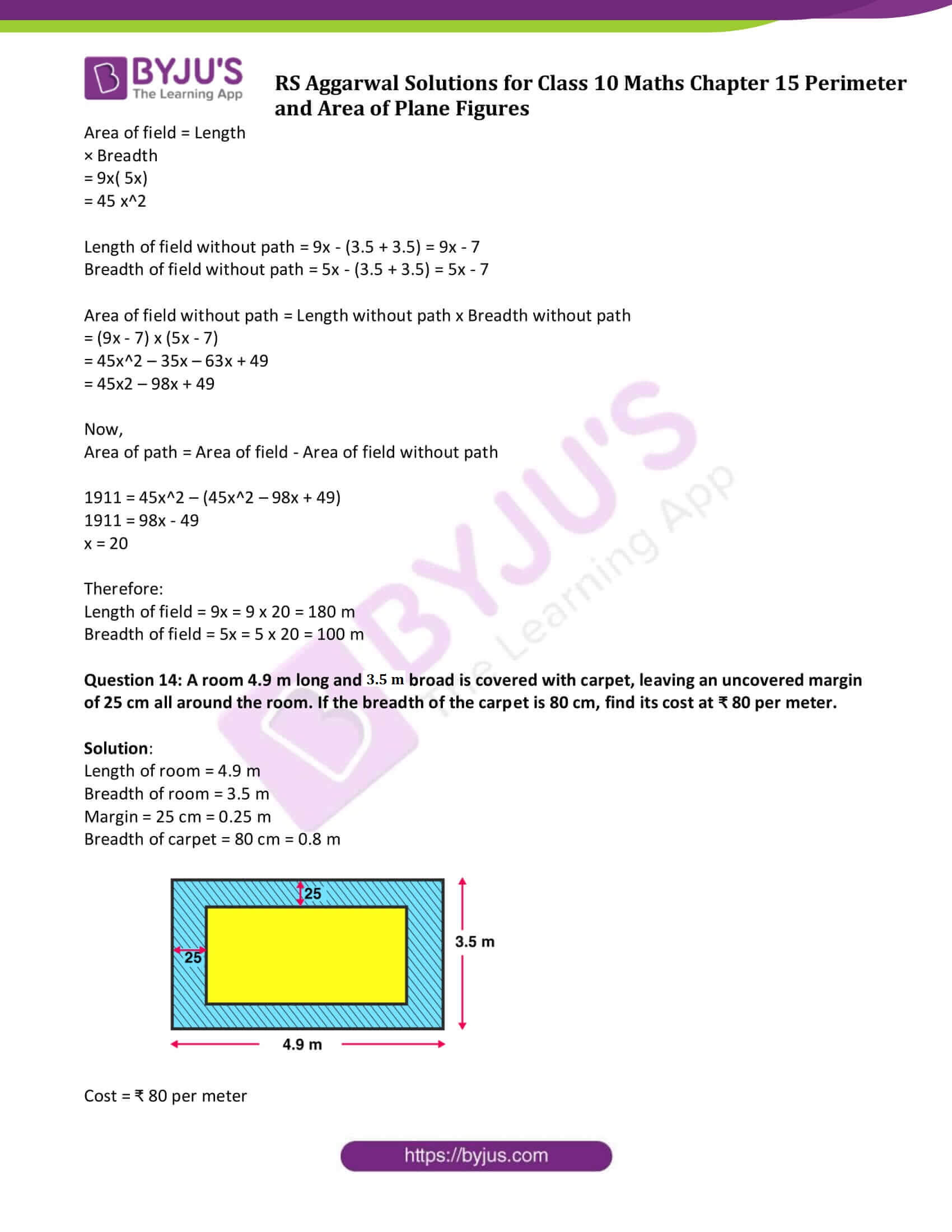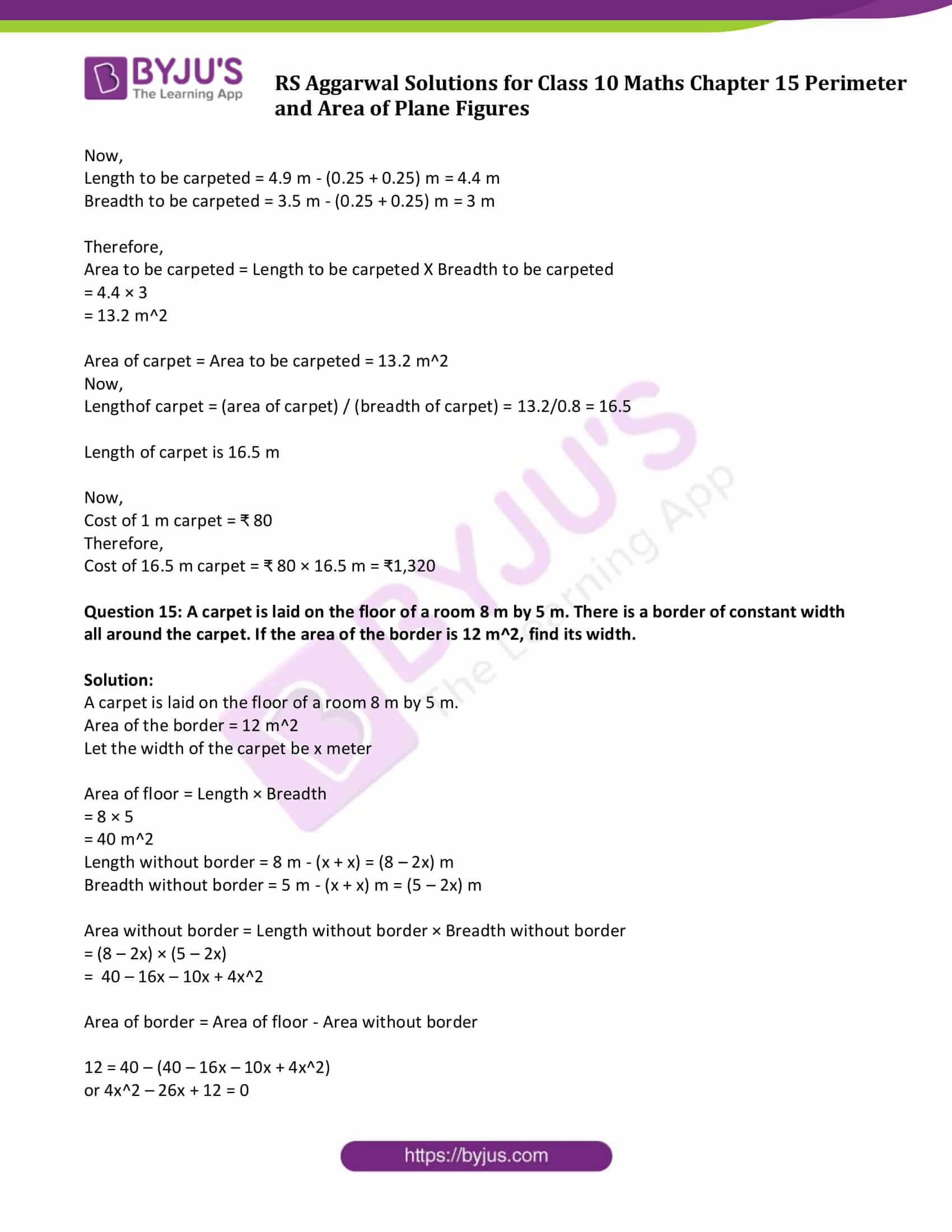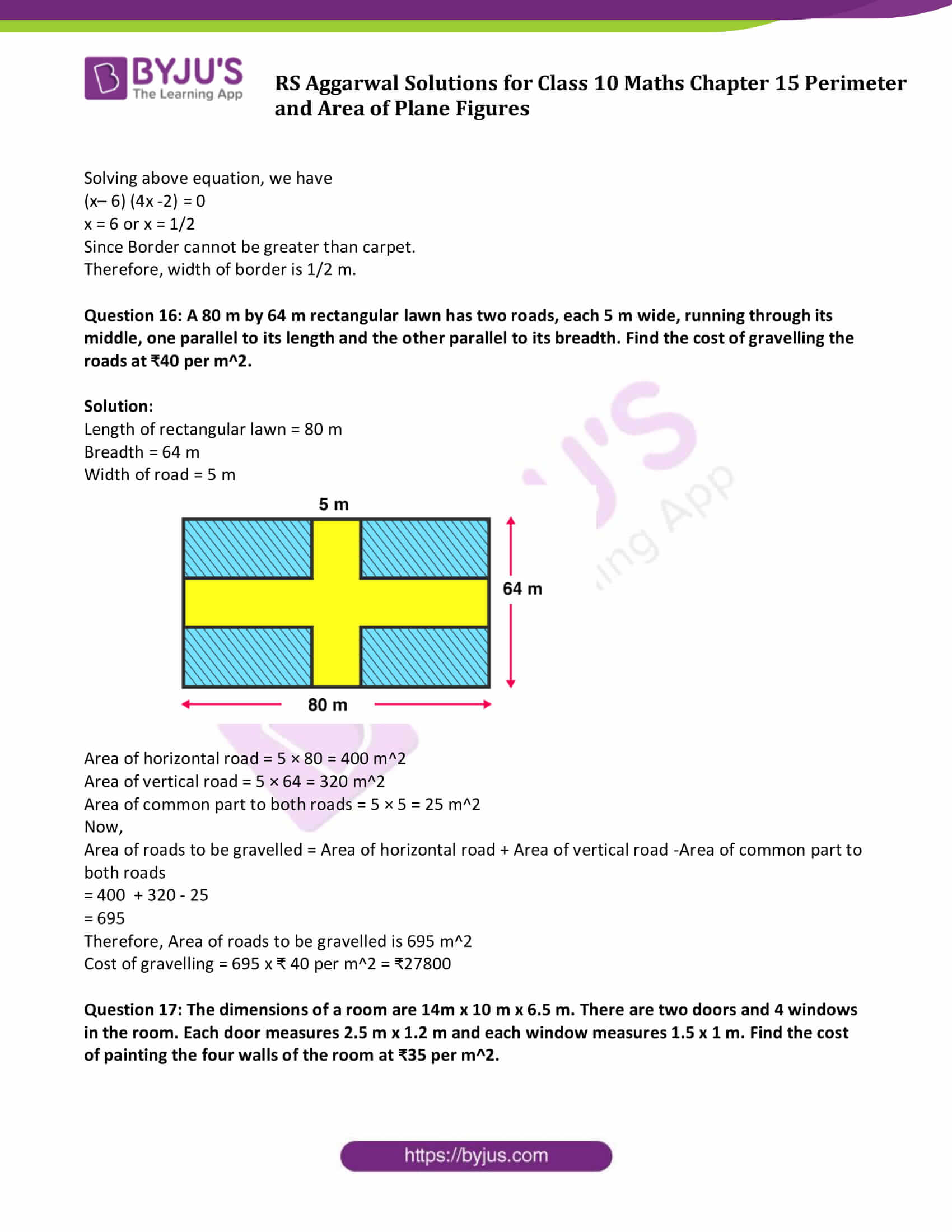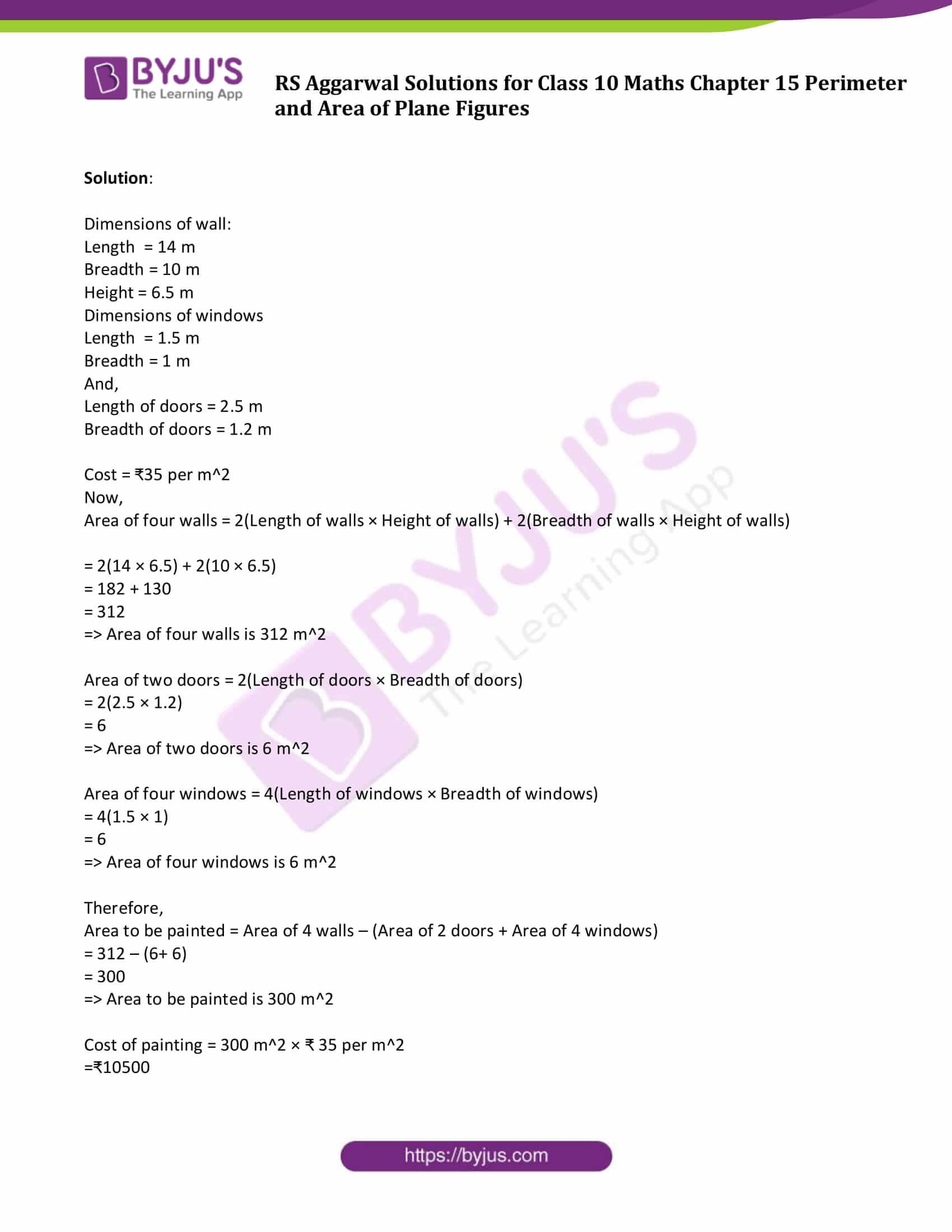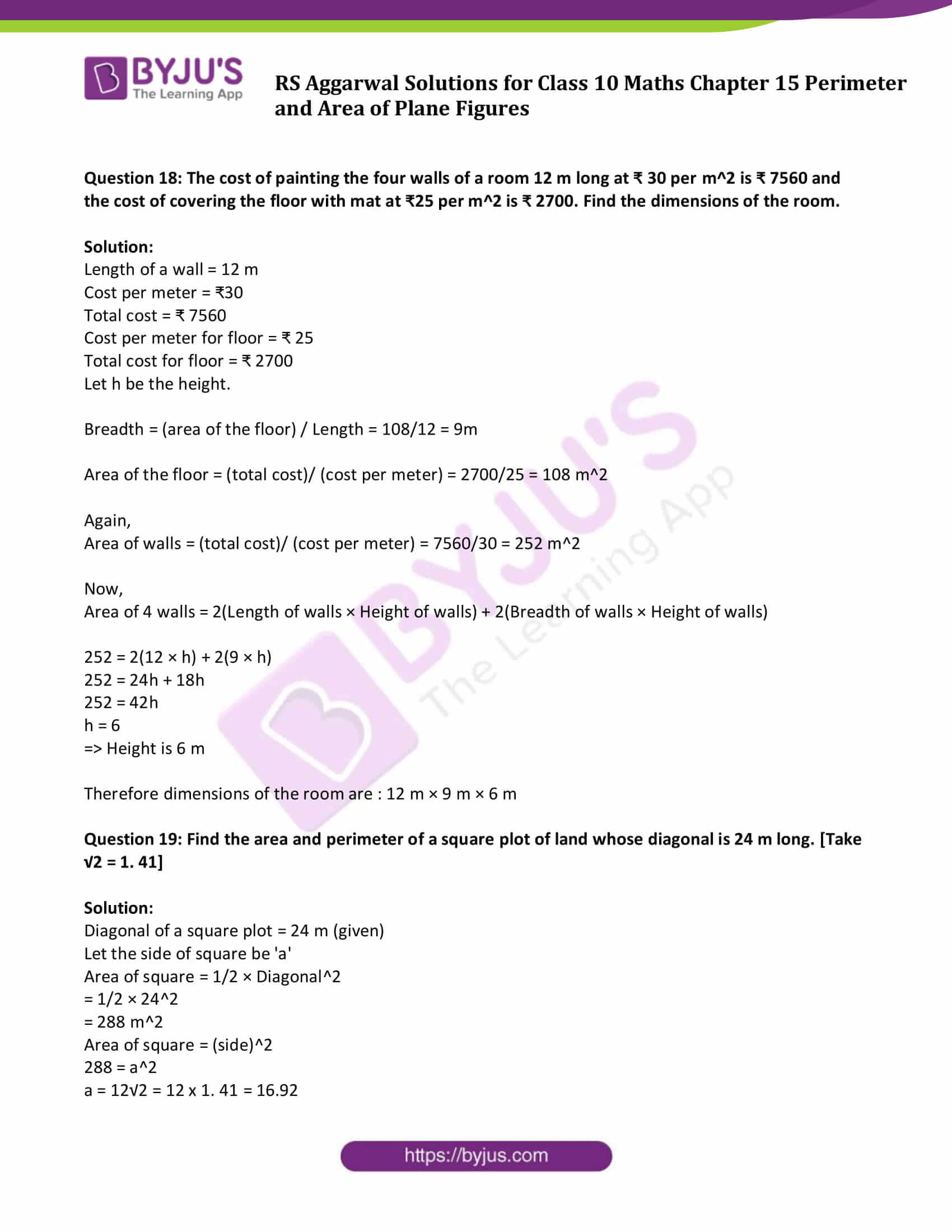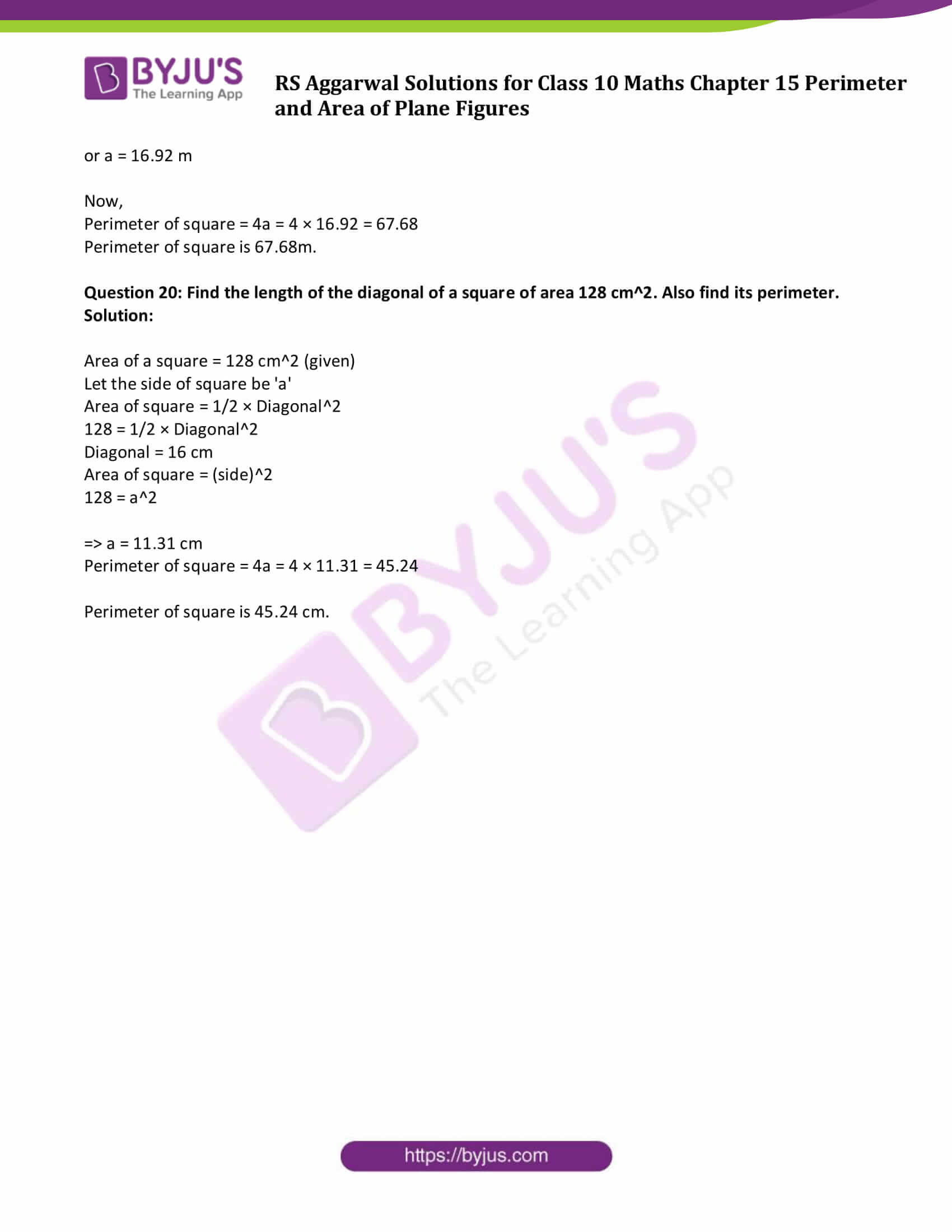### Access Answers to Maths R S Aggarwal Chapter 15 – Perimeter and Area of Plane Figures

Get detailed solutions for all the questions listed under the below exercises:

Exercise 15 A Solutions

Exercise 15 B Solutions

## Exercise 15A

Question 1: Find the area of the triangle whose base measures 24 cm and the corresponding height measures 14.5 cm.

Solution:

Given:

Base of triangle = 24 cm

Height = 14.5 cm

Area of a triangle = 1/2 x Base X Height

= 1/2 x 24 x 14.5

= 174

Area of a triangle is 174 cm2.

Question 2: Find the area of the triangle whose sides are 42 cm, 34 cm and 20 cm in length. Find the height corresponding to the longest side.

Solution: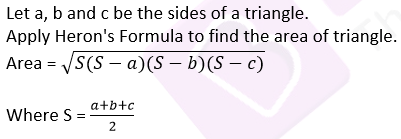Here a = 42 cm, b = 34 cm and c = 20 cm

S = (42 +34 +20)/2 = 48

Area = √(48(48-42)(48-34)(48-20))

= √(48 x 6 x 14 x 28)

= 336

Area of triangle is 336 cm2.

Clearly,

Length of longest side = 42 cm

Also we know, Area of a triangle = 1/2 × Base × Height

336 = 1/2 × 42 × Height

336 = 21 x Height

or Height = 16

The height corresponding to the longest side is 16 cm.

Question 3: Find the area of the triangle whose sides are 18 cm, 24 cm and 30 cm. Also, find the height corresponding to the smallest side.

Solution:Here a = 18 cm, b = 24 cm, c = 30 cm

Now,

S = 1/2(18+24+30) = 36

Area = √(36(36-18)(36-24)(36-30))

= √(36 × 18× 12 × 6)

= 216

Area is 216 cm2

From given, Length of smallest side = 18 cm

Area of a triangle = 1/2 × Base × Height

216 = 1/2 x 18 x height

Height = 24

Therefore, the height corresponding to the smallest side is 24 cm.

Question 4: The sides of a triangle are in the ratio 5: 12 : 13, and its perimeter is 150 m. Find the area of the triangle.

Solution:

Perimeter of triangle = 150 m (given)

Let the sides of triangle be, a, b and c,

On dividing 150 m in the ratio 5 : 12 : 13, we get

a = 5x cm

b = 12x cm

c = 13x cm

We know that, Perimeter of a triangle = Sum of all the sides = a + b + c

150 = 5x + 12x + 13x

= 30 x

x = 5

Sides are:

a = 5x = 25 cm

b = 12x = 60 cm

c = 13x = 65 cm

Now,S = 1/2(25+60+65) = 75 cm

Area = √(75(75-25)(75-60)(75-65))

= √(75 × 50 × 15 × 10)

= 750

Area of triangle is 750 cm2.

Question 5: The perimeter of a triangular field is 540 m, and its sides are in the ratio 25:17:12. Find the area of the field. Also, find the cost of ploughing the field at ₹40 per 100 m2.

Solution:

Perimeter of triangle = 540 m

Let the sides of triangle be, a, b and c

On dividing 540 m in the ratio 25:17:12, we get

a = 25x m

b = 17x m

c = 12x m

We know that, Perimeter of a triangle = Sum of all the sides = a + b + c

540 = 25x + 17x + 12x

= 54 x

x = 10

Sides are:

a = 25x = 250 m

b = 17x = 170 m

c = 12x = 120 mS = 1/2(250+170+120) = 270 m

Area = √(270(270-250)(270-170)(270-120))

= √(270 x 20 x 10 x 150)

= 9000

Area of triangle is 9000 m2.

Now,

The cost of ploughing 100 m2 = ₹40

The cost of ploughing 1 m2 = ₹ 40/100

Therefore, cost of ploughing 9000 m2 = 9000 x 40/100 = ₹3600

Question 6: The perimeter of a right triangle is 40 cm and its hypotenuse measures 17 cm. Find the area of the triangle.

Solution:

The perimeter of a right triangle = 40 cm

Hypotenuse measures = 17 cm.

Let x and y are the another sides.

40 = 17 + x + y

x + y = 23

or y = 23 – y

So, we have 3 sides as x, 23-x and 17.

Apply Pythagoras theorem:

Hypotenuse2 = Base2 + Perpendicular2

172 = x2 + (23-x)2

289 = x2 + 529 + x2 – 46x

x2 – 23x + 120 = 0

(x – 8)(x – 15) = 0

x = 8 or x = 15

If x = 8 then y = 23-8 = 15

If x = 15 then y = 23-15 = 8

Now,

Area of triangle = 1/2 × base × height

= 1/2 × 8 × 15

= 60

Therefore, Area of triangle is 60 cm2

Question 7: The difference between the sides at right angle in a right-angled triangle is 7 cm. The area of the triangle is 60 cm2. Find its perimeter.

Solution:

Let x cm be the one of the sides, then (x – 7) cm be another side.

Area of triangle = 60 cm2 (given)

We know, Area of triangle = 1/2(Base x height)

60 = 1/2(x (x – 7))

120 = x2 – 7x

or x2 – 7x – 120 = 0

Solving above equation, we have

(x – 15)(x + 8) = 0

x = 15 or x = -8

Since length measure cannot be negative, so neglect x = -8

One side = 15 cm

Another Side = x – 7 = 15 – 7 = 8 cm

Apply Pythagoras theorem:

Hypotenuse2 = Base2 + Perpendicular2

Hypotenuse2 = √(152 + 82)

Hypotenuse = √289 = 17

Therefore, perimeter of triangle = Sum of all the sides = (15 + 8 + 17) cm = 40 cm

Question 8: The lengths of the two sides of a right triangle containing the right angle differ by 2 cm. If the area of the triangle is 24 cm2, find the perimeter of the triangle.

Solution:

Let x cm be the one of the sides, then (x – 2) cm be another side.

Area of triangle = 24 cm2 (given)

We know, Area of triangle = 1/2(Base x height)

24 = 1/2(x (x – 2))

48 = x2 – 2x

or x2 – 2x – 48 = 0

Solving above equation, we have

(x + 6)(x – 8) = 0

x = -6 or x = 8

Since length measure cannot be negative, so neglect x = -6

One side = 8 cm

Another Side = x – 2 = 8 – 2 = 6 cm

Apply Pythagoras theorem:

Hypotenuse2 = Base2 + Perpendicular2

Hypotenuse2 = √(82 + 62)

Hypotenuse = √100 = 10

Therefore, perimeter of triangle = Sum of all the sides = (6 + 8 + 10) cm = 24 cm

Question 9: Each side of an equilateral triangle is 10 cm. Find

(i) the area of the triangle and (ii) the height of the triangle.

Solution:

Side of an equilateral triangle = 10 cm

(i) Area of a triangle = √3/4 (side)2 = √3/4 x 10 x 10 = 43.3

Area of a triangle is 43.3 cm2

(ii) Height of the triangle = √3/2 (side) = √3/2 x 10 = 8.66

Height of the triangle is 8.66 cm

Question 10: The height of an equilateral triangle is 6 cm. Find its area. [Take √3 = 1 .73]

Solution:

Height of an equilateral triangle = 6 cm

Let x be a side of triangle.

Height = √3/2 (side) = √3/2 x

6 = √3/2 x

x = 12/√3

x = 12√3/3 = 6.92 cm

Now,

Area of a triangle = √3/4 (side)2 = √3/4 x 6.92 x 6.92 = 20.76 cm2

Question 11: If the area of an equilateral triangle is 36√3 cm2, find its perimeter.

Solution:

Let each side of an equilateral triangle be “a” cm

Area of an equilateral triangle = 36√3 cm2 (Given)

Area of an equilateral triangle = √3/4 (side)2

36√3 = √3/4 x a2

a2 = 144

or a = 12 cm

Perimeter = 3 (side) = 3 x 12 = 36 cm.

Question 12: If the area of an equilateral triangle is 81√3 cm2, find its height.

Solution:

Let each side of an equilateral triangle be “a” cm

Area of an equilateral triangle = 81√3 cm2 (Given)

Area of an equilateral triangle = √3/4 (side)2

81√3 = √3/4 x a2

a2 = 324

or a = 18 cm

Now,

Height of an equilateral triangle = √3/2 (side) = √3/4 (18) = 9√3 cm.

Perimeter = 3 (side) = 3 x 12 = 36 cm.

Question 13: The base of a right-angled triangle measures 48 cm and its hypotenuse measures 50 cm. Find the area of the triangle.

Solution:

We are given with a right-angled triangle whose measures are

Base = 48 cm

Hypotenuse = 50 cm

Using Pythagoras Theorem:

Hypotenuse2 = Base2 + Perpendicular2

502 = 482 + Perpendicular2

or Perpendicular2 = 2500 – 2304

or Perpendicular = 14 cm

Now,

Area of a triangle = 1/2 × Base × Height

= 1/2 × 48 cm × 14 cm

= 336 cm2

Question 14: The hypotenuse of a right-angled triangle is 65 cm and its base is 60 cm. Find the length of perpendicular and the area of the triangle.

Solution:

We are given with a right-angled triangle whose measures are

Base = 6 m

Hypotenuse = 6.5 m

Using Pythagoras Theorem:

Hypotenuse2 = Base2 + Perpendicular2

(6.5)2 = (6)2 + Perpendicular2

or Perpendicular2 = 42.25 – 36

or Perpendicular = 2.5 m

Now,

Area of a triangle = 1/2 × Base × Height

= 1/2 × 6 m × 2.5 m

= 7.5 cm2

Question 15: Find the area of a right-angled triangle, the radius of whose circumcircle measure 8 cm and the altitude drawn to the hypotenuse measures 6 cm.

Solution:

Radius of circle = 8 cm and

Altitude = 6 cm

Since, in a right-angled triangle the hypotenuse is the diameter of circumcircle. (given)

= 2 × 8

= 16

=> Hypotenuse = 16 cm

Area of a triangle = 1/2 × Base × Height

(Consider hypotenuse as base and the altitude to the hypotenuse as height)

= 1/2 × 16 x 6

= 48

=> Area of a triangle is 48 cm2

Question 16: Find the length of the hypotenuse of an isosceles right-angled triangle whose area is 200 cm2. Also, find its perimeter. [Given: √2 = 1.41]

Solution:

Let two sides of an isosceles right-angled triangle are of measure “a”.

Area of triangle = 200 cm2. (given)

200 = 1/2(a2)

=> a = 20 cm

Now,

Hypotenuse = √(a2+a2) = √(2a2) = √2 a

= 20 √2

= 20 x 1.414

= 28.28

=> Hypotenuse is 28.28 cm

Perimeter of triangle = Sum of all the sides = 2a + Hypotenuse

= 40 + 28.28

= 68.28

=> Perimeter of triangle is 68.28 cm.

Question 17: The base of an isosceles triangle measures 80 cm and its area is 360 cm2. Find the perimeter of the triangle.

Solution:

Let two sides of an isosceles triangle are of measure “a”.

Base = b = 80 cm

Area of triangle = 360 cm2. (given)

We know, Area of isosceles triangle = 1/4 × b√(4a2 – b2)

360 = 1/4 x 80 x √(4a2 – (80)2)

360 = 20 x √(4a2 – 6400)

360 = 20 x 2√(a2 – 1600)

9 = √(a2 – 1600)

Squaring both sides

81 = a2 – 1600

a = 41

=> a = 41 cm

Perimeter of triangle = Sum of all the sides = 41 + 41 + 80 = 162

Therefore, the perimeter of triangle is 162 cm.

Question 18: Each of the equal sides of an isosceles triangle measures 2 cm more than its height, and the base of the triangle measures 12 cm. Find the area of the triangle.

Solution:

Let h be the height, then

Each of the equal sides of an isosceles triangle = (h + 2) cm and

Base = 12 cm

Area of a triangle = 1/2 × Base × Height

= 1/2 x 12 x h ….(1)

Area of isosceles triangle = 1/4 x b x √(4a2 – b2)

= 1/2 x 12 x √(4(h+2)2 – 144) …(2)

From (1) and (2), we get

1/2 x 12 x h = 1/2 x 12 x √(4(h+2)2 – 144)

6h = 3√(4h2 + 16h + 16-144)

2h = √(4h2 + 16h-128)

4h2 = 4h2 + 16h – 128

16h = 128

or h = 8

Height is 8 cm.

Now,

Area of a triangle = 1/2 × Base × Height

= 1/2 × 12 cm × 8 cm

= 1/2 × 96 cm2

= 48 cm2

Question 19: Find the area and perimeter of an isosceles right triangle, each of whose equal sides measures 10 cm. [Given: √2 = 1 .41]

Solution:

Equal sides of measure = 10 cm

Here, base and perpendicular are equal sides.

Area of a triangle = 1/2 × Base × Height

= 1/2 x 10 x 10

= 50

From Pythagorean Theorem:

Hypotenuse2 = Base2 + Perpendicular2

= 102 + 102

= 200

or Hypotenuse = 10 v2 cm = 10 x 1 .41 cm = 14.1 cm

Perimeter = Sum of all the sides = 10 + 10 + 14.1 = 34.1

Therefore, Area of required triangle is 50 cm2 and perimeter = 34.1 cm

Question 20: In the given figure, ∆ABC is an equilateral triangle the length of whose side is equal to 10 cm, and ∆DBC is right-angled at D and BD = 8 cm. Find the area of the shaded region. [Take √3 = 1.732.]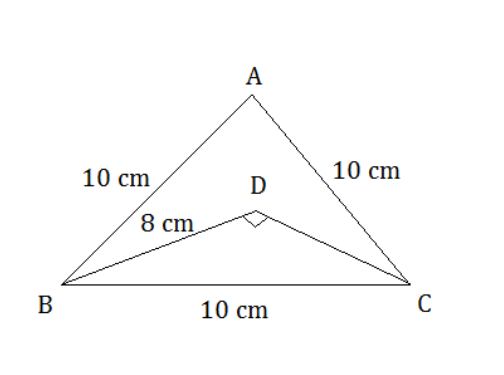Solution:∆ABC is an equilateral triangle the length of whose side is equal to 10 cm, and ∆DBC is right-angled at D and BD = 8 cm.

From figure:

Area of shaded region = Area of ABC – Area of DBC …..(1)

Area of △ABC:

Area = v3/4 (side)2 = v3/4 (10)2 = 43.30

So area of △ABC is 43.30 cm2

Area of right △DBC:

Area = 1/2 x base x height …(2)

From Pythagorean Theorem:

Hypotenuse2 = Base2 + Height2

BC2 = DB2 + Height2

100 – 64 = Height2

36 = Height2

or Height = 6

equation (2) =>

Area = 1/2 x 8 x 6 = 24

So area of △DBC is 24 cm2

Equation (1) implies

Area of shaded region = 43.30 – 24 = 19.30

Therefore, Area of shaded region = 19.3 cm2

## Exercise 15B

Question 1: The perimeter of a rectangular plot of land is 80 m and its breadth is 16 m. Find the length and area of the plot.

Solution:

Perimeter of rectangle = 80 m

Let x be the length and 16m breadth.

We know, perimeter = 2 [length + breadth]

75 = 2(x + 16)

75 = (2x + 32)

Or x = 21.5

Thus,

Length of the rectangle is 21. 5 meter

Again,

Area of the rectangular plot = Length X Breadth

= (16 × 21.5)

= 344

So, Area of the rectangular plot is 344 cm2

Therefore, length of rectangle is 21.5 m and the area is 344 m2.

Question 2: The length of a rectangular park is twice its breadth, and its perimeter measures 840 m. Find the area of the park.

Solution:

The length of a rectangular park = 2(its breadth)

Perimeter = 840 m (Given)

Let x be the breadth of a rectangular park then, length of a rectangular park is 2x m.

We know, Perimeter of rectangle = 2(Length + Breadth)

840 = 2(2x + x)

840 = 6x

or x = 140

Breadth = x = 140 m

and Length = 2x = 2 x 140 = 280 m

Area of a rectangular park = Length x Breadth = 140 x 280 = 39200 m2.

Question 3: One side of a rectangle is 12 cm long and its diagonal measures 37 cm. Find the other side and the area of the rectangle.

Solution:

Let ABCD be the rectangle in which AB

Let length of rectangle = 12 cm and diagonal= 37 m

Let breadth be the other side.

By Pythagoras theorem,

= v(49 x 25)

= v(1225)

Thus, length = 12 cm and breadth = 35 cm

Area of rectangle = (12 × 35) = 420

Hence, the other side is 35 cm and the area of rectangle is 420 cm2.

Question 4: The area of a rectangular plot is 462 m2 and its length is 28 m. Find the perimeter of the plot.

Solution:

Area of a rectangular plot = 462 m2

Length of rectangle = 28 m

Let x m be the breadth of the plot

Now,

Area = Length × Breadth = (28x)

462 = 28x

or x = 16.5

Again,

Perimeter of the plot = 2(length + breadth) = 2(28 + 16.5) = 89

Therefore,

Breadth of plot = 16. 5 m

Perimeter of the plot = 89 m

Question 5: A lawn is in the form of a rectangle whose sides are in the ratio 5 : 3. The area of the lawn is 3375 m2. Find the cost of fencing the lawn at ₹65 per metre.

Solution:

Cost of fencing lawn = ₹ 65 per metre.

Area of lawn = 3375 m2

Length = 5x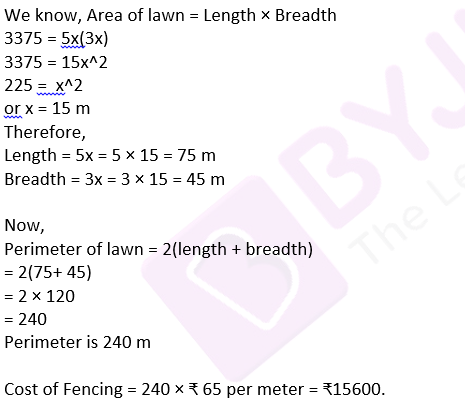Question 6: A room is 16 m long and 13.5 m broad. Find the cost of covering its floor with 75-cm-wide carpet at ₹60 per metre.

Solution:

Cost of covering room floor = ₹60 per metre.

Length of room = 16 m

Breadth of room = 13.5 m

Breadth of carpet = 75 cm = 0.75 m

We know, Area of room = Length × Breadth

= 16 × 13.5

= 216

⇨ Area of room is 216 m2

Length of carpet can be calculated by using below formula:

Length of carpet = (area of room)/(Breadth of carpet)

= 216/0.75

= 288 m

Now,

Cost of covering the floor = 288 m × ₹60 per meter = ₹17280

Question 7: The floor of a rectangular hall is 24 m long and 18 m wide. How many carpets, each of length 2.5 m and breadth 80 cm, will be required to cover the floor of the hall?

Solution:

Length of hall = 24 m

Breadth of hall = 18 m

Length of carpet = 2.5 m

Breadth of carpet = 80 cm = 0.8 m

Area of hall = Length × Breadth

= 24 × 18

= 432 m2

And, Area of carpet = Length × Breadth

= 2.5 × 0.8

= 2 m2

Number of carpets = (area of hall)/(Area of carpet) = 432/2 = 216

That is, number of carpets are 216.

Question 8: A 36 m-long, 15m-broad verandah is to be paved with stones, each measuring 6 dm by 5 dm. How many stones will be required?

Solution:

Length of verandah = 36 m

Breadth of verandah = 15 m

Length of stones = 6 dm = 0.6 m

Breadth of stones = 5 dm = 0.5 m

Now,

Area of verandah = Length × Breadth

= 36 x 15

= 540

Area = 540 m2

Area of stones = Length × Breadth

= 0.6× 0.5

= 0.3

Area of stone = 0.3 m2

Number of stones = (area of verandah)/(Area of stones) = 540/0.3 = 1800

That is, number of stones required are 1800.

Question 9: The area of a rectangle is 192 cm2 and its perimeter is 56 cm. Find the dimensions of the rectangle.

Solution:

The area of a rectangle is 192 cm2 and its perimeter is 56 cm.

let l cm be the length and b cm be the breadth.

Area of rectangle = Length × Breadth

192 = l × b

⇨ l = 192/b ….(1)

Perimeter of rectangle = 2(length + breadth)

56 = 2(l + b)

from (1)

56 = 2(192/b + b)

28 = (192 + b2)/b

b2– 28b + 192 = 0

(b – 12 )(b – 16 ) = 0

b = 12 cm or b = 16 cm

Choose b = 12cm then l = 16 cm

Hence, Length is 16 cm and Breadth is 12 cm.

Question 10: A rectangular park 35 m long 18 m wide is to be covered with grass, leaving 2.5 m uncovered all around it. Find the area to be laid with grass.

Solution:

Given:

Length of the park = 35 m

Breadth of the park = 18 m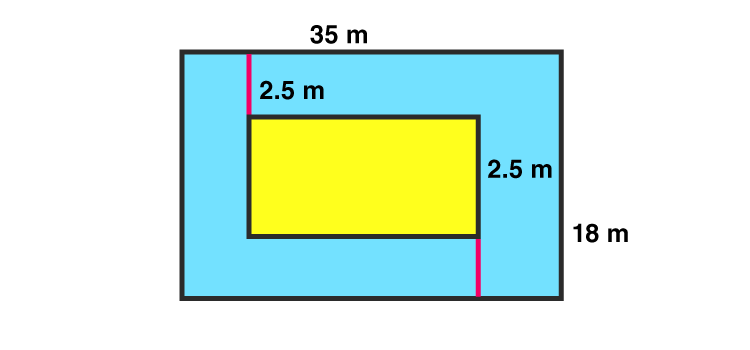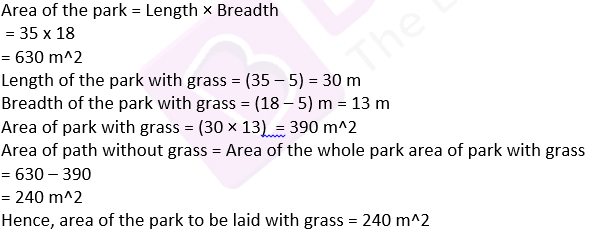Question 11: A rectangular plot measures 125m by 78m. It has gravel path 3m wide all around on the outside. Find the area of the path and the cost of gravelling it at ₹ 75 per m2.

Solution:

Given:

Length of the plot = 125 m

Breadth of the plot = 78 m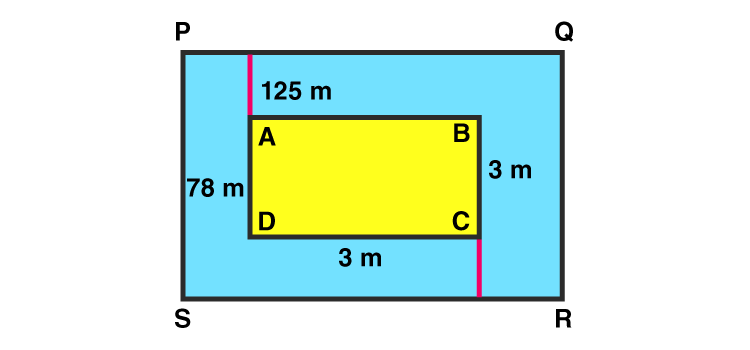Area of plot ABCD = Length × Breadth

= 125 x 78

= 9750 m2

Length of the plot including the path= (125 + 3 + 3) m = 131 m

Breadth of the plot including the path = (78 + 3 + 3) m = 84 m

Now,

Area of plot PQRS including the path = Length × Breadth

= (131 × 84)

= 11004 m2

Area of path = Area of plot PQRS – Area of plot ABCD

= 11004 – 9750

= 1254 m2

Cost of gravelling = ₹75 per m2

Cost of gravelling the whole path = ₹ (1254 × 75) = ₹ 94050

Hence, cost of gravelling the path is ₹ 94050.

Question 12: (i) A footpath of uniform width runs all around the inside of a rectangular field 54 m long and 35m wide. If the area of the path is 420 m2, find the width of the path.

Solution:

Given:

Length of field = 54 m

Breadth of field = 35 m

Let x m be the width of the path.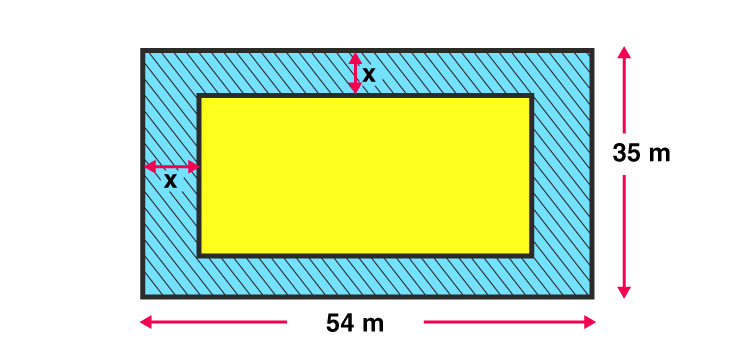Area of field = Length × Breadth

= 54 x 35

= 1890 m2

Length of field without path = 54 – (x + x) = 54 – 2x

Breadth of field without path = 35 – (x + x) = 35 – 2x

Now,

Area of field without path = Length without path × Breadth without path

= (54 – 2x)(35 – 2x)

= 1890 – 70x – 108x + 4x2

= 4x2 – 178x + 1890 …(1)

Area of path = Area of field – Area of field without path

420 = 1890 – (1890 – 178x + 4x2)

(Using equation (1))

420 = 178x – 4x2

or 4x2 – 178x + 420 = 0

or 2x2 – 89x + 210 = 0

On solving above equation, we have

x = 42 or x = 5/2 m = 2.5 m

As width of park cannot be more than breadth of field, so width of park is 2.5 m.

(ii) A carpet is laid on the floor of a room 8m by 5m. There is a border of constant width all around the carpet. If the area of the border is 12 m2, find its width.

Solution:

Area of border = 12 m2

Length of room = 8m

Let x be the width of carpet

Length of carpet = 8-x-x = (8-2x)m

Breadth of carpet = 5-x-x = (5-2x)m

Area of room (carpet + border) = 8 x 5 = 40 m2

Area of carpet = (8-2x)(5-2x)

= (40-16x-10x+4x2)

= (4x2-26x+40)

Now,

Area of ground = Area of border + Area of carpet

40 = 12 + 4x2-26x+40

2x2-13x+6 = 0

After solving above equation, we have

x = 1/2 or x = 6 (ignore as it is longer than room)

So, Width of border = 0.5m

Question 13: The length and the breadth of a rectangular garden are in the ratio 9 : 5. A path 3.5 m wide, running all around inside it has an area of 1911 m2. Find the dimensions of the garden.

Solution:

Let the length and breadth of a rectangular garden be 9x and 5x.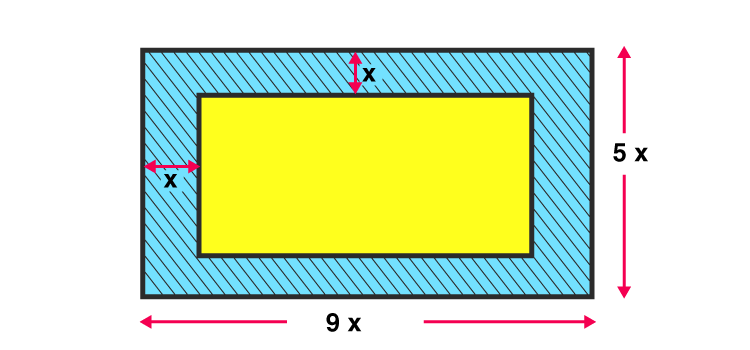Area of field = Length × Breadth

= 9x( 5x)

= 45 x2

Length of field without path = 9x – (3.5 + 3.5) = 9x – 7

Breadth of field without path = 5x – (3.5 + 3.5) = 5x – 7

Area of field without path = Length without path x Breadth without path

= (9x – 7) x (5x – 7)

= 45x2 – 35x – 63x + 49

= 45×2 – 98x + 49

Now,

Area of path = Area of field – Area of field without path

1911 = 45x2 – (45x2 – 98x + 49)

1911 = 98x – 49

x = 20

Therefore:

Length of field = 9x = 9 x 20 = 180 m

Breadth of field = 5x = 5 x 20 = 100 m

Question 14: A room 4.9 m long and 3.5 m broad is covered with carpet, leaving an uncovered margin of 25 cm all around the room. If the breadth of the carpet is 80 cm, find its cost at ₹ 80 per meter.

Solution:

Length of room = 4.9 m

Breadth of room = 3.5 m

Margin = 25 cm = 0.25 m

Breadth of carpet = 80 cm = 0.8 m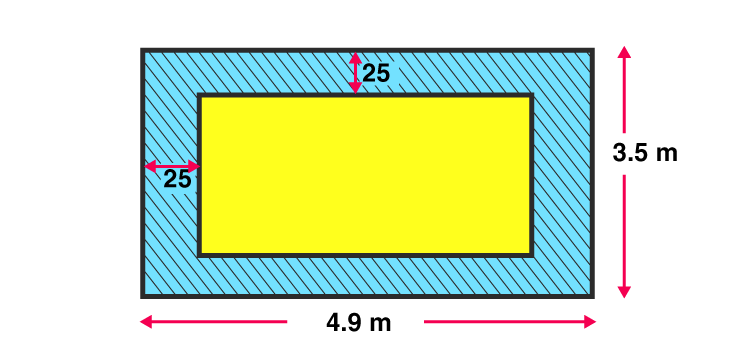Cost = ₹ 80 per meter

Now,

Length to be carpeted = 4.9 m – (0.25 + 0.25) m = 4.4 m

Breadth to be carpeted = 3.5 m – (0.25 + 0.25) m = 3 m

Therefore,

Area to be carpeted = Length to be carpeted X Breadth to be carpeted

= 4.4 × 3

= 13.2 m2

Area of carpet = Area to be carpeted = 13.2 m2

Now,

Lengthof carpet = (area of carpet) / (breadth of carpet) = 13.2/0.8 = 16.5

Length of carpet is 16.5 m

Now,

Cost of 1 m carpet = ₹ 80

Therefore,

Cost of 16.5 m carpet = ₹ 80 × 16.5 m = ₹1,320

Question 15: A carpet is laid on the floor of a room 8 m by 5 m. There is a border of constant width all around the carpet. If the area of the border is 12 m2, find its width.

Solution:

A carpet is laid on the floor of a room 8 m by 5 m.

Area of the border = 12 m2

Let the width of the carpet be x meter

Area of floor = Length × Breadth

= 8 × 5

= 40 m2

Length without border = 8 m – (x + x) = (8 – 2x) m

Breadth without border = 5 m – (x + x) m = (5 – 2x) m

Area without border = Length without border × Breadth without border

= (8 – 2x) × (5 – 2x)

= 40 – 16x – 10x + 4x2

Area of border = Area of floor – Area without border

12 = 40 – (40 – 16x – 10x + 4x2)

or 4x2 – 26x + 12 = 0

Solving above equation, we have

(x– 6) (4x -2) = 0

x = 6 or x = 1/2

Since Border cannot be greater than carpet.

Therefore, width of border is 1/2 m.

Question 16: A 80 m by 64 m rectangular lawn has two roads, each 5 m wide, running through its middle, one parallel to its length and the other parallel to its breadth. Find the cost of gravelling the roads at ₹40 per m2.

Solution:

Length of rectangular lawn = 80 m

Width of road = 5 m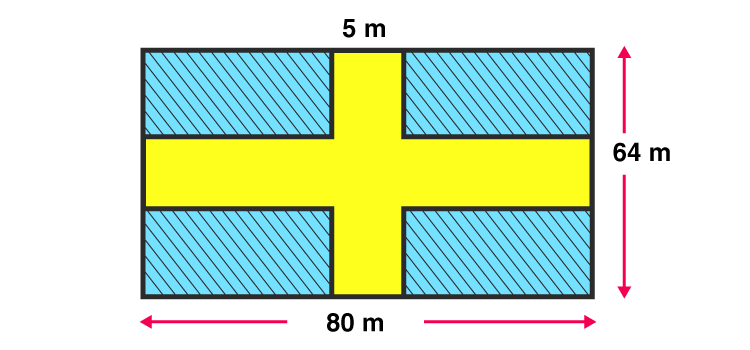Area of horizontal road = 5 × 80 = 400 m2

Area of vertical road = 5 × 64 = 320 m2

Area of common part to both roads = 5 × 5 = 25 m2

Now,

Area of roads to be gravelled = Area of horizontal road + Area of vertical road -Area of common part to both roads

= 400 + 320 – 25

= 695

Therefore, Area of roads to be gravelled is 695 m2

Cost of gravelling = 695 x ₹ 40 per m2 = ₹27800

Question 17: The dimensions of a room are 14m x 10 m x 6.5 m. There are two doors and 4 windows in the room. Each door measures 2.5 m x 1.2 m and each window measures 1.5 x 1 m. Find the cost of painting the four walls of the room at ₹35 per m2.

Solution:

Dimensions of wall:

Length = 14 m

Height = 6.5 m

Dimensions of windows

Length = 1.5 m

And,

Length of doors = 2.5 m

Breadth of doors = 1.2 m

Cost = ₹35 per m2

Now,

Area of four walls = 2(Length of walls × Height of walls) + 2(Breadth of walls × Height of walls)

= 2(14 × 6.5) + 2(10 × 6.5)

= 182 + 130

= 312

⇨ Area of four walls is 312 m2

Area of two doors = 2(Length of doors × Breadth of doors)

= 2(2.5 × 1.2)

= 6

⇨ Area of two doors is 6 m2

Area of four windows = 4(Length of windows × Breadth of windows)

= 4(1.5 × 1)

= 6

⇨ Area of four windows is 6 m2

Therefore,

Area to be painted = Area of 4 walls – (Area of 2 doors + Area of 4 windows)

= 312 – (6+ 6)

= 300

⇨ Area to be painted is 300 m2

Cost of painting = 300 m2 × ₹ 35 per m2

=₹10500

Question 18: The cost of painting the four walls of a room 12 m long at ₹ 30 per m2 is ₹ 7560 and the cost of covering the floor with mat at ₹25 per m2 is ₹ 2700. Find the dimensions of the room.

Solution:

Length of a wall = 12 m

Cost per meter = ₹30

Total cost = ₹ 7560

Cost per meter for floor = ₹ 25

Total cost for floor = ₹ 2700

Let h be the height.

Breadth = (area of the floor) / Length = 108/12 = 9m

Area of the floor = (total cost)/ (cost per meter) = 2700/25 = 108 m2

Again,

Area of walls = (total cost)/ (cost per meter) = 7560/30 = 252 m2

Now,

Area of 4 walls = 2(Length of walls × Height of walls) + 2(Breadth of walls × Height of walls)

252 = 2(12 × h) + 2(9 × h)

252 = 24h + 18h

252 = 42h

h = 6

⇨ Height is 6 m

Therefore dimensions of the room are : 12 m × 9 m × 6 m

Question 19: Find the area and perimeter of a square plot of land whose diagonal is 24 m long. [Take √2 = 1. 41]

Solution:

Diagonal of a square plot = 24 m (given)

Let the side of square be ‘a’

Area of square = 1/2 × Diagonal2

= 1/2 × 242

= 288 m2

Area of square = (side)2

288 = a2

a = 12√2 = 12 x 1. 41 = 16.92

or a = 16.92 m

Now,

Perimeter of square = 4a = 4 × 16.92 = 67.68

Perimeter of square is 67.68m.

Question 20: Find the length of the diagonal of a square of area 128 cm2. Also find its perimeter.

Solution:

Area of a square = 128 cm2 (given)

Let the side of square be ‘a’

Area of square = 1/2 × Diagonal2

128 = 1/2 × Diagonal2

Diagonal = 16 cm

Area of square = (side)2

128 = a2

⇨ a = 11.31 cm

Perimeter of square = 4a = 4 × 11.31 = 45.24

Perimeter of square is 45.24 cm.

## R S Aggarwal Solutions for Class 10 Maths Chapter 15 Perimeter and Area of Plane Figures

In this chapter students will study important concepts on perimeter and area of plane geometrical figures as listed below:

• Perimeter of Plane Figures
• Area of Plane Figures
• Important Formulas List

### Key Features of R S Aggarwal Solutions for Class 10 Maths Chapter 15 Perimeter and Area of Plane Figures

1. Students will learn how to find area and perimeter of a plane geometrical figures with the help of various solved problems.

2. Easy and simple language is used.

3. All questions are solved with the help of diagram for better understanding on Perimeter and Area of Plane Figures.